# Tag: topos

A month ago, Stephen Wolfram put out a little booklet (140 pages) What Is ChatGPT Doing … and Why Does It Work?.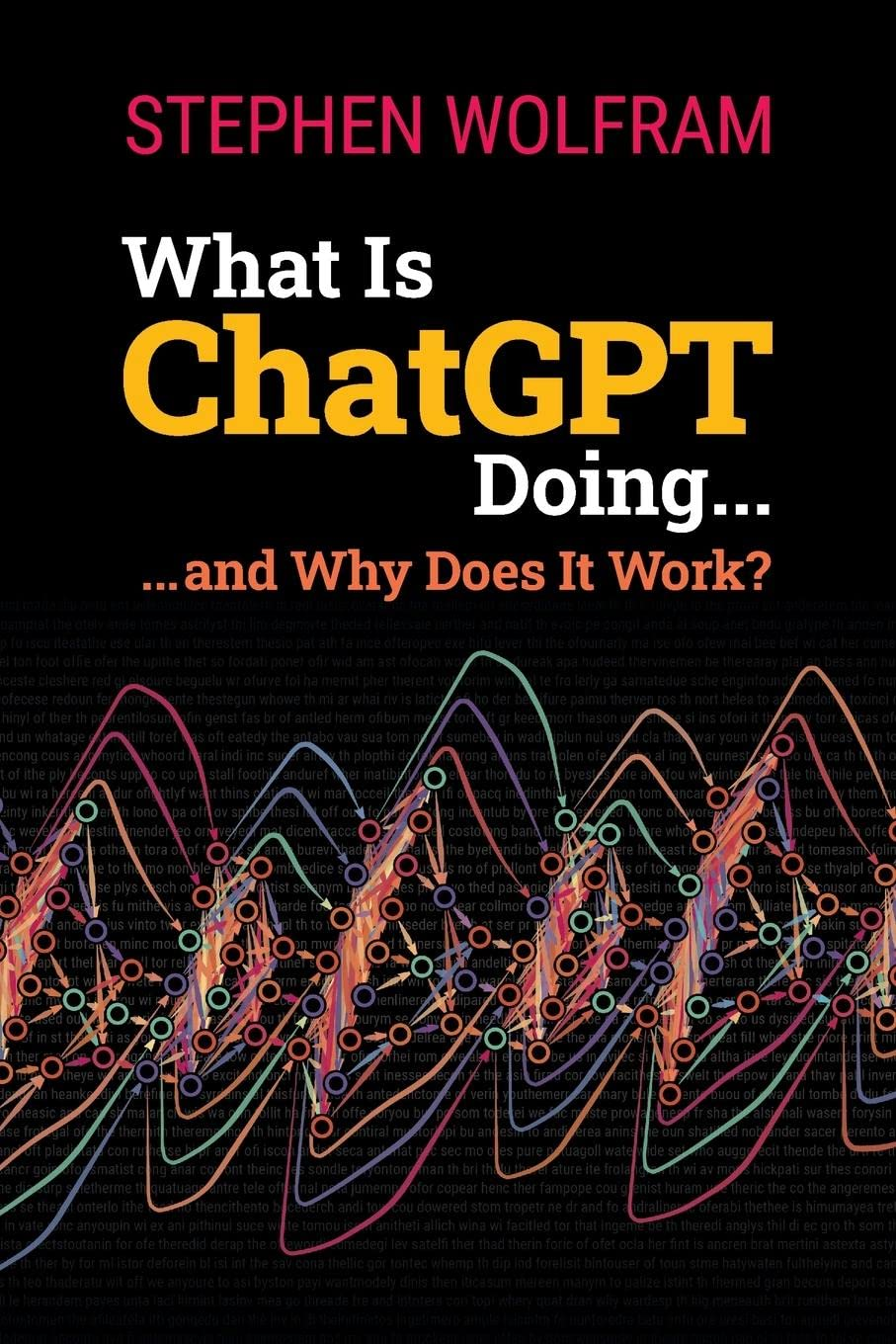It gives a gentle introduction to large language models and the architecture and training of neural networks.

The entire book is freely available:

The advantage of these online texts is that you can click on any of the images, copy their content into a Mathematica notebook, and play with the code.

This really gives a good idea of how an extremely simplified version of ChatGPT (based on GPT-2) works.

Downloading the model (within Mathematica) uses about 500Mb, but afterwards you can complete any prompt quickly, and see how the results change if you turn up the ‘temperature’.

You should’t expect too much from this model. Here’s what it came up with from the prompt “The major results obtained by non-commutative geometry include …” after 20 steps, at temperature 0.8:

 NestList[StringJoin[#, model[#, {"RandomSample", "Temperature" -> 0.8}]] &, "The major results obtained by non-commutative geometry include ", 20]

 

The major results obtained by non-commutative geometry include vernacular accuracy of math and arithmetic, a stable balance between simplicity and complexity and a relatively low level of violence. 

Lol.

In the more philosophical sections of the book, Wolfram speculates about the secret rules of language that ChatGPT must have found if we want to explain its apparent succes. One of these rules, he argues, must be the ‘logic’ of languages:

But is there a general way to tell if a sentence is meaningful? There’s no traditional overall theory for that. But it’s something that one can think of ChatGPT as having implicitly “developed a theory for” after being trained with billions of (presumably meaningful) sentences from the web, etc.

What might this theory be like? Well, there’s one tiny corner that’s basically been known for two millennia, and that’s logic. And certainly in the syllogistic form in which Aristotle discovered it, logic is basically a way of saying that sentences that follow certain patterns are reasonable, while others are not.

Something else ChatGPT may have discovered are language’s ‘semantic laws of motion’, being able to complete sentences by following ‘geodesics’:

And, yes, this seems like a mess—and doesn’t do anything to particularly encourage the idea that one can expect to identify “mathematical-physics-like” “semantic laws of motion” by empirically studying “what ChatGPT is doing inside”. But perhaps we’re just looking at the “wrong variables” (or wrong coordinate system) and if only we looked at the right one, we’d immediately see that ChatGPT is doing something “mathematical-physics-simple” like following geodesics. But as of now, we’re not ready to “empirically decode” from its “internal behavior” what ChatGPT has “discovered” about how human language is “put together”.

So, the ‘hidden secret’ of successful large language models may very well be a combination of logic and geometry. Does this sound familiar?

If you prefer watching YouTube over reading a book, or if you want to see the examples in action, here’s a video by Stephen Wolfram. The stream starts about 10 minutes into the clip, and the whole lecture is pretty long, well over 3 hours (about as long as it takes to read What Is ChatGPT Doing … and Why Does It Work?).

In the shape of languages we started from a collection of notes, made a poset of text-snippets from them, and turned this into an enriched category over the unit interval $[0,1]$, following the paper paper An enriched category theory of language: from syntax to semantics by Tai-Danae Bradley, John Terilla and Yiannis Vlassopoulos.

This allowed us to view the text-snippets as points in a Lawvere pseudoquasi metric space, and to define a ‘topos’ of enriched presheaves on it, including the Yoneda-presheaves containing semantic information of the snippets.

In the previous post we looked at ‘building a second brain’ apps, such as LogSeq and Obsidian, and hoped to use them to test the conjectured ‘topos of the unconscious’.

In Obsidian, a vault is a collection of notes (with their tags and other meta-data), together with all links between them.

The vault of the language-poset will have one note for every text-snipped, and have a link from note $n$ to note $m$ if $m$ is a text-fragment in $n$.

In their paper, Bradley, Terilla and Vlassopoulos use the enrichment structure where $\mu(n,m) \in [0,1]$ is the conditional probablity of the fragment $m$ to be extended to the larger text $n$.

Most Obsidian vaults are a lot more complicated, possibly having oriented cycles in their internal link structure.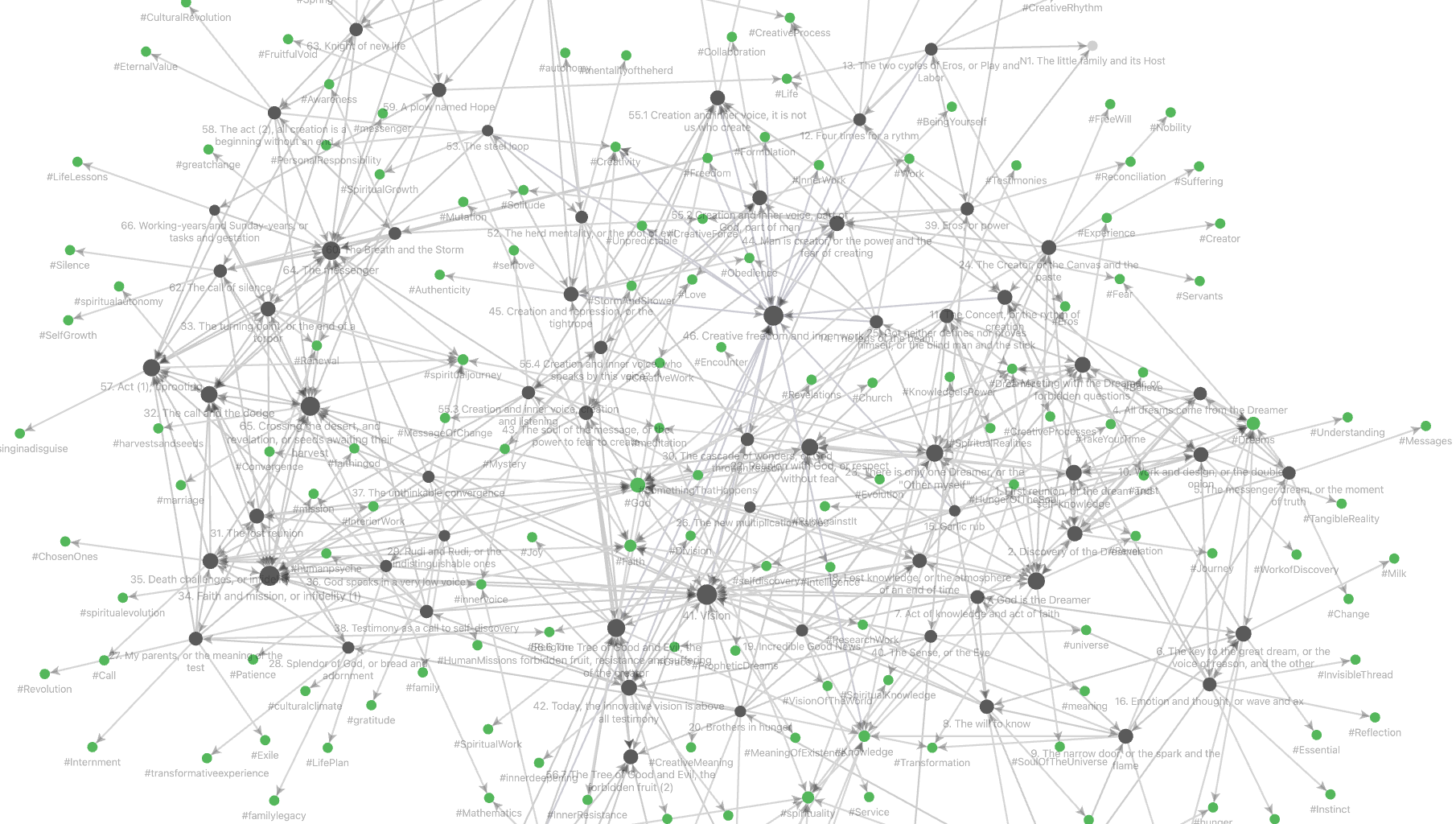Still, it is always possible to turn the notes of the vault into a category enriched over $[0,1]$, in multiple ways, depending on whether we want to focus on the internal link-structure or rather on the semantic similarity between notes, or any combination of these.

Let $X$ be a set of searchable data from your vault. Elements of $X$ may be

• words contained in notes
• in- or out-going links between notes
• tags used
• YAML-frontmatter

Assign a positive real number $r_x \geq 0$ to every $x \in X$. We see $r_x$ as the ‘relevance’ we attach to the search term $x$. So, it is possible to emphasise certain key-words or tags, find certain links more important than others, and so on.

For this relevance function $r : X \rightarrow \mathbb{R}_+$, we have a function defined on all subsets $Y$ of $X$

$$f_r~:~\mathcal{P}(X) \rightarrow \mathbb{R}_+ \qquad Y \mapsto f_r(Y) = \sum_{x \in Y} r_x$$

Take a note $n$ from the vault $V$ and let $X_n$ be the set of search terms from $X$ contained in $n$.

We can then define a (generalised) Jaccard distance for any pair of notes $n$ and $m$ in $V$:

$$d_r(n,m) = \begin{cases} 0~\text{if f_r(X_n \cup X_m)=0} \\ 1-\frac{f_r(X_n \cap X_m)}{f_r(X_n \cup X_m)}~\text{otherwise} \end{cases}$$

This distance is symmetric, $d_r(n,n)=0$ for all notes $n$, and the crucial property is that it satisfies the triangle inequality, that is, for all triples of notes $l$, $m$ and $n$ we have

$$d_r(l,n) \leq d_r(l,m)+d_r(m,n)$$

For a proof in this generality see the paper A note on the triangle inequality for the Jaccard distance by Sven Kosub.

How does this help to make the vault $V$ into a category enriched over $[0,1]$?

The poset $([0,1],\leq)$ is the category with objects all numbers $a \in [0,1]$, and a unique morphism $a \rightarrow b$ between two numbers iff $a \leq b$. This category has limits (infs) and colimits (sups), has a monoidal structure $a \otimes b = a \times b$ with unit object $1$, and an internal hom

$$Hom_{[0,1]}(a,b) = (a,b) = \begin{cases} \frac{b}{a}~\text{if b \leq a} \\ 1~\text{otherwise} \end{cases}$$We say that the vault is an enriched category over $[0,1]$ if for every pair of notes $n$ and $m$ we have a number $\mu(n,m) \in [0,1]$ satisfying for all notes $n$

$$\mu(n,n)=1~\quad~\text{and}~\quad~\mu(m,l) \times \mu(n,m) \leq \mu(n,l)$$

for all triples of notes $l,m$ and $n$.

Starting from any relevance function $r : X \rightarrow \mathbb{R}_+$ we define for every pair $n$ and $m$ of notes the distance function $d_r(m,n)$ satisfying the triangle inequality. If we now take

$$\mu_r(m,n) = e^{-d_r(m,n)}$$

then the triangle inequality translates for every triple of notes $l,m$ and $n$ into

$$\mu_r(m,l) \times \mu_r(n,m) \leq \mu_r(n,l)$$

That is, every relevance function makes $V$ into a category enriched over $[0,1]$.

Two simple relevance functions, and their corresponding distance and enrichment functions are available from Obsidian’s Graph Analysis community plugin.

To get structural information on the link-structure take as $X$ the set of all incoming and outgoing links in your vault, with relevance function the constant function $1$.

‘Jaccard’ in Graph Analysis computes for the current note $n$ the value of $1-d_r(n,m)$ for all notes $m$, so if this value is $a \in [0,1]$, then the corresponding enrichment value is $\mu_r(m,n)=e^{a-1}$.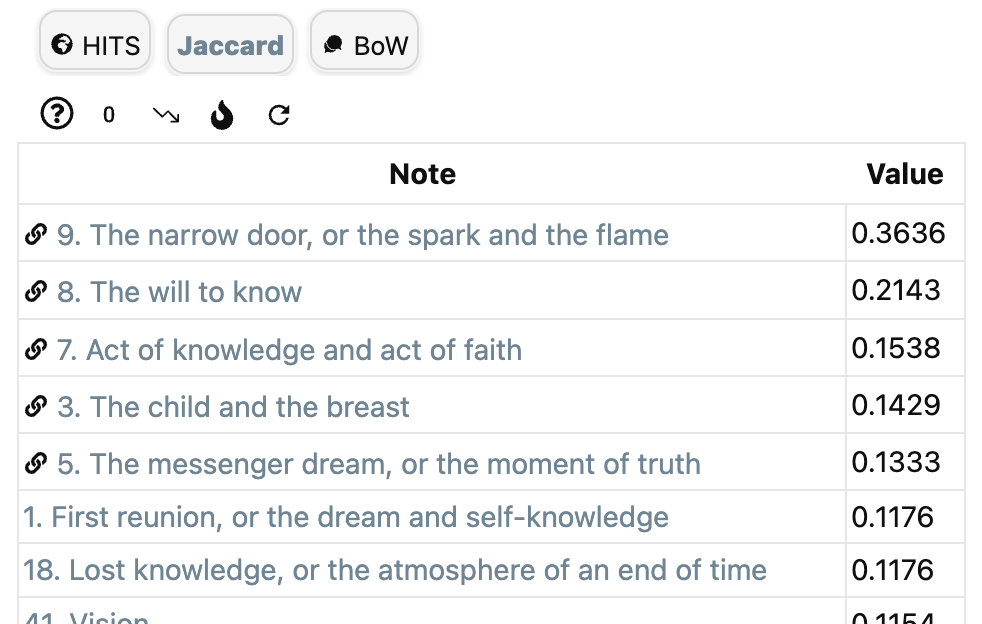To get semantic information on the similarity between notes, let $X$ be the set of all words in all notes and take again as relevance function the constant function $1$.

To access ‘BoW’ (Bags of Words) in Graph Analysis, you must first install the (non-community) NLP plugin which enables various types of natural language processing in the vault. The install is best done via the BRAT plugin (perhaps I’ll do a couple of posts on Obsidian someday).

If it gives for the current note $n$ the value $a$ for a note $m$, then again we can take as the enrichment structure $\mu_r(n,m)=e^{a-1}$.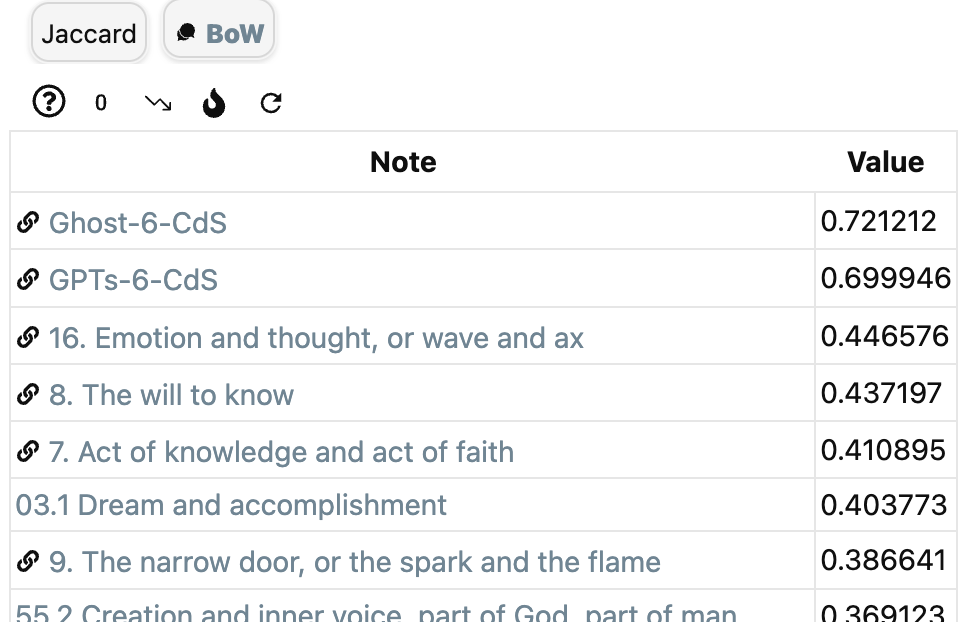Graph Analysis offers more functionality, and a good introduction is given in this clip:

Calculating the enrichment data for custom designed relevance functions takes a lot more work, but is doable. Perhaps I’ll return to this later.

Mathematically, it is probably more interesting to start with a given enrichment structure $\mu$ on the vault $V$, describe the category of all enriched presheaves $\widehat{V_{\mu}}$ and find out what we can do with it.

(tbc)

Previously in this series:

Next:

The super-vault of missing notes

Before ChatGPT, the hype among productivity boosters was a PKMs or Personal knowledge management system.

It gained popularity through Tiago Forte’s book ‘Building a second brain’, and (for academics perhaps a more useful read) ‘How to take smart notes’ by Sönke Ahrens.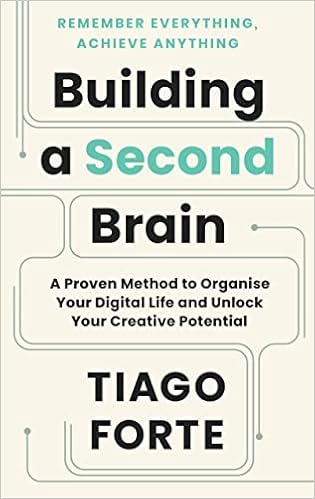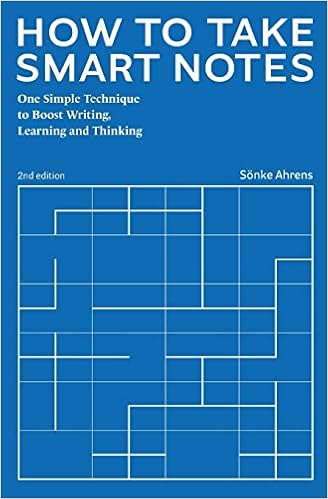These books promote new techniques for note-taking (and for storing these notes) such as the PARA-method, the CODE-system, and Zettelkasten.

Unmistakable Creative has some posts on the principles behing the ‘second brain’ approach.

Your brain isn’t like a hard drive or a dropbox, where information is stored in folders and subfolders. None of our thoughts or ideas exist in isolation. Information is organized in a series of non-linear associative networks in the brain.

Networked thinking is not just a more efficient way to organize information. It frees your brain to do what it does best: Imagine, invent, innovate, and create. The less you have to remember where information is, the more you can use it to summarize that information and turn knowledge into action.

and

A network has no “correct” orientation and thus no bottom and no top. Each individual, or “node,” in a network functions autonomously, negotiating its own relationships and coalescing into groups. Examples of networks include a flock of birds, the World Wide Web, and the social ties in a neighborhood. Networks are inherently “bottom-up” in that the structure emerges organically from small interactions without direction from a central authority.

-Tiago Forte, Tagging for Personal Knowledge Management

There are several apps you can use to start building your second brain, the more popular seem to be Roam Research, LogSeq, and Obsidian.

These systems allow you to store, link and manipulate a large collection of notes, query them as a database, modify them in various ways via plugins or scripts, and navigate the network created via graph-views.

Exactly the kind of things we need to modify the simple system from the shape of languages-post into a proper topos of the unconscious.

I’ve been playing around with Obsidian which I like because it has good LaTeX plugins, powerful database tools via the Dataview plugin, and one can execute codeblocks within notes in almost any programming language (python, haskell, lean, Mathematica, ruby, javascript, R, …).

Most of all it has a vibrant community of users, an excellent forum, and a well-documented Obsidian hub.

There’s just one problem, I’m a terrible note-taker, so how can I begin to load my ‘second brain’?

Obsidian has several plugins to import data, such as your Kindle highlights, your Twitter feed, your Readwise-data, and many others, but having been too lazy in the past, I cannot use any of them.

In fact, the only useful collection of notes I have are my blog-posts. So, I’ve uploaded NeverEndingBooks into Obsidian, one note per post (admittedly, not very Zettelkasten-like), half a million words in total.

Fortunately, I did tag most of these posts at the time. Together with other meta-data this results in the Graph view below (under ‘Files’ toggled tags, under ‘Groups’ three tag-colours, and under ‘Display’ toggled arrows). One can add colour-groups based on tags or other information (here, red dots are posts tagged ‘Grothendieck’, the blue ones are tagged ‘Conway’, the purple ones tagged ‘Connes’, just for the sake of illustration). In Obsidian you can zoom into this graph, place a pointer on a node to highlight the connecting dots, and much more.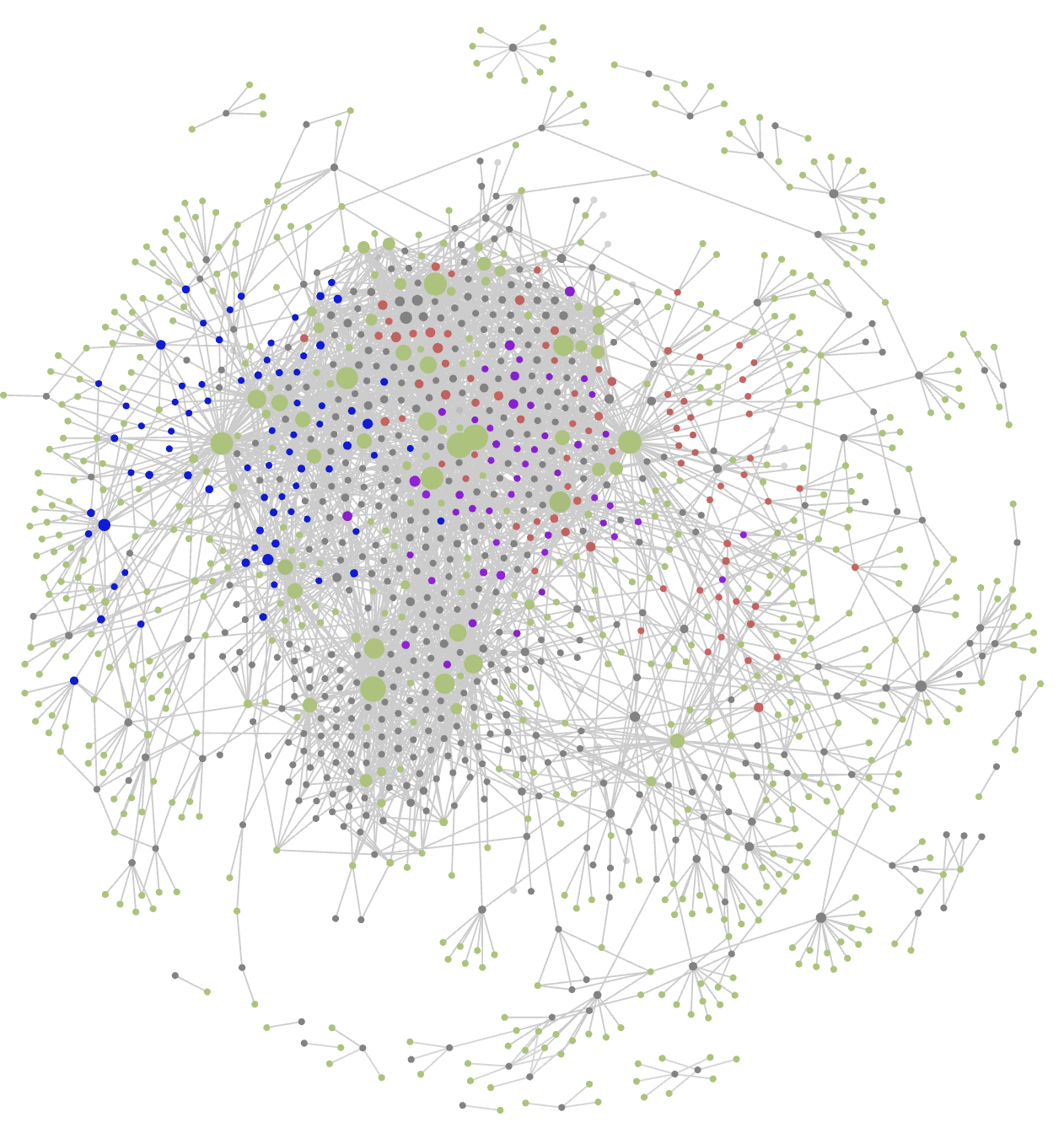Because I tend to forget such things, and as it may be useful to other people running a WordPress-blog making heavy use of MathJax, here’s the procedure I followed:

1. Follow the instructions from Convert wordpress articles to markdown.

In the wizard I’ve opted to go only for yearly folders, to prefix posts with the date, and to save all images.

2. This gives you a directory with one folder per year containing markdown versions of your posts, and in each year-folder a subfolder ‘img’ containing all images.

Turn this directory into an Obsidian-vault by opening Obsidian, click on the ‘open another vault’ icon (third from bottom-left), select ‘Open folder as vault’ and navigate to your directory.

3. You will notice that most of your LaTeX cannot be parsed because during the markdown-process backslashes are treaded as special character, resulting in two backslashes for every LaTeX-command…

A remark before trying to solve this: another option might be to use the wordpress-to-hugo-exporter, resulting in clean LaTeX, but lacking the possibility to opt for yearly-folders (it dumps all posts into one folder), and it makes a mess of the image-files.

4. So, we will need to do a lot of search&replaces in all files, and need a convenient tool for this.

First option was the Sublime Text app, which is free and does the search&replaces quickly. The problem is that you have to save each of the files, one at a time! This may take hours.

I’ve done it using the Search and Replace app (3$) which allows you to make several searches/replaces at the same time (I messed up LaTeX code in previous exports, so needed to do many more changes). It warns you that it is dangerous to replace strings in all files (which is the reason why Sublime Text makes it difficult), you can ignore it, but only after you put the ‘img’ folders away in a safe place. Otherwise it will also try to make the changes to these files, recognise that they are not text-files, and drop them altogether… That’s it. I now have a backup network-version of this blog.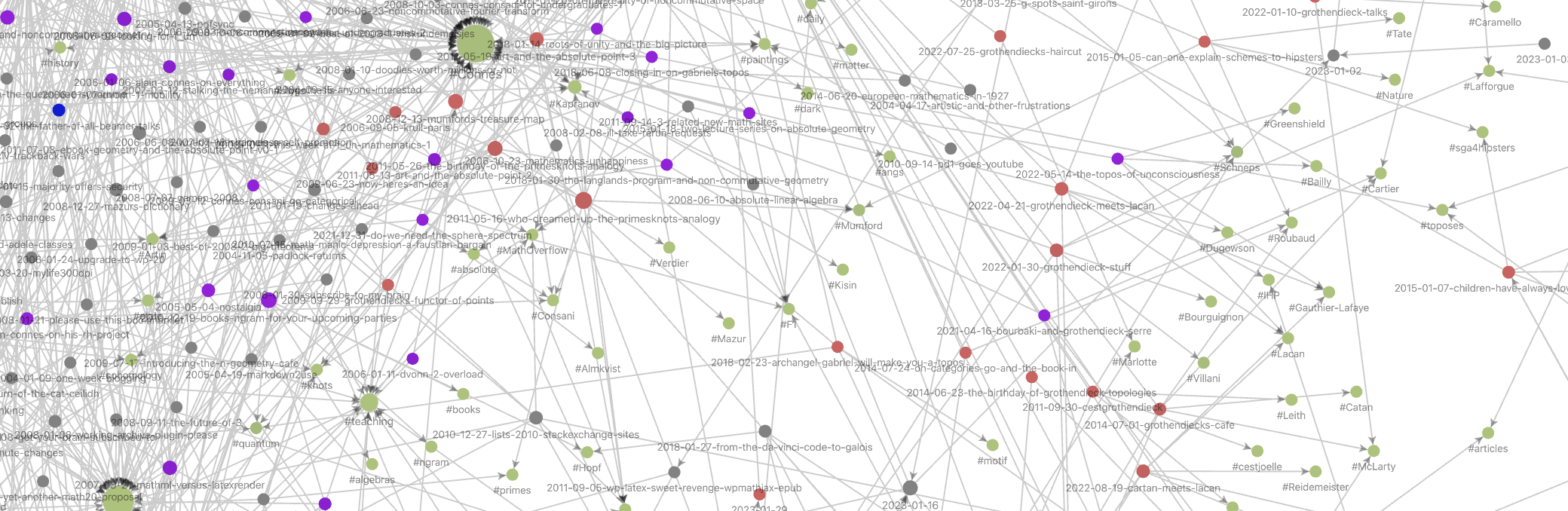As we mentioned in the previous post a first attempt to construct the ‘topos of the unconscious’ might be to start with a collection of notes (the ‘conscious’) and work on the semantics of text-snippets to unravel (a part of) the unconscious underpinning of these notes. We also mentioned that the poset-structure in that post should be replaced by a more involved network structure. What interests me most is whether such an approach might be doable ‘in practice’, and Obsidian looks like the perfect tool to try this out. What we need is a sufficiently large set of notes, of independent interest, to inject into Obsidian. The more meta it is, the better… (tbc) Previously in this series: Next: The enriched vault In the topology of dreams we looked at Sibony’s idea to view dream-interpretations as sections in a fibered space. The ‘points’ in the base-space and fibers consisting of chunks of text, perhaps connected by links. The topology and shape of this fibered space is still shrouded in mystery. Let’s look at a simple approach to turn a large number of texts into a topos, and define a loose metric on it. There’s this paper An enriched category theory of language: from syntax to semantics by Tai-Danae Bradley, John Terilla and Yiannis Vlassopoulos. Tai-Danae Bradley is an excellent communicator of everything category related, so probably it is more fun to read her own blogposts on this paper: or to watch her Categories for AI talk: ‘Category Theory Inspired by LLMs’: Let’s start with a collection of notes. In the paper, they consider all possible texts written in some language, but it may be a set of webpages to train a language model, or a set of recollections by someone. Next, shred these notes into chunks of text, and point one of these to all the texts obtained by deleting some words at the start and/or end of it. For example, the note ‘a red rose’ will point to ‘a red’, ‘red rose’, ‘a’, ‘red’ and ‘rose’ (but not to ‘a rose’). You may call this a category, to me it is just as a poset$(\mathcal{L},\leq)$. The maximal elements are the individual words, the minimal elements are the notes, or websites, we started from.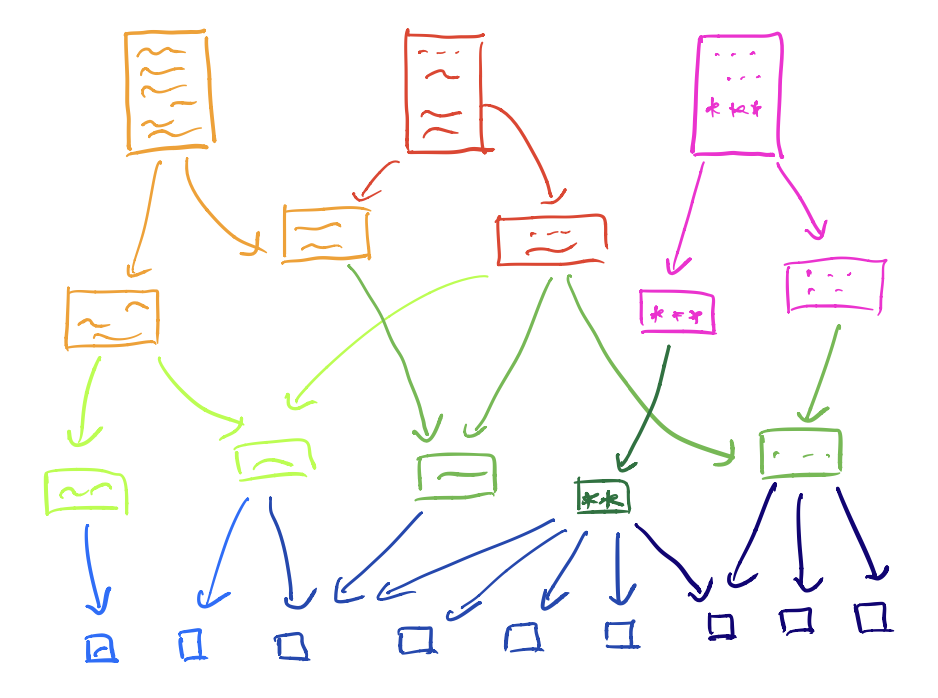A down-set$A$of this poset$(\mathcal{L},\leq)$is a subset of$\mathcal{L}$closed under taking smaller elements, that is, if$a \in A$and$b \leq a$, then$b \in A$. The intersection of two down-sets is again a down-set (or empty), and the union of down-sets is again a downset. That is, down-sets define a topology on our collection of text-snippets, or if you want, on language-fragments. For example, the open determined by the word ‘red’ is the collection of all text-fragments containing this word. The corresponding presheaf topos$\widehat{\mathcal{L}}$is then just the category of all (set-valued) presheaves on this topological space. As an example, the Yoneda-presheaf$\mathcal{Y}(p)$of a text-snippet$p$is the contra-variant functor $$(\mathcal{L},\leq) \rightarrow \mathbf{Sets}$$ sending any$q \leq p$to the unique map$\ast$from$q$to$p$, and if$q \not\leq p$then we map it to$\emptyset$. If$A$is a down-set (an open of over topological space) then the sections of$\mathcal{Y}(p)$over$A$are$\{ \ast \}$if for all$a \in A$we have$a \leq p$, and$\emptyset$otherwise. The presheaf$\mathcal{Y}(p)$already contains some semantic information about the snippet$p$as it gives all contexts in which$p$appears. Perhaps interesting is that the ‘points’ of the topos$\widehat{\mathcal{L}}$are the notes we started from. Recall that Connes and Gauthier-Lafaey want to construct a topos describing someone’s unconscious, and points of that topos should be the connection with that person’s consciousness. Suppose you want to unravel your unconscious. You start by writing down a large set of notes containing all relevant facts of your life. Then you construct from these notes the above collection of snippets and its corresponding pre-sheaf topos. Clearly, you wrote your notes consciously, but probably the exact phrasing of these notes, or recurrent themes in them, or some text-combinations are ruled by your unconscious. Ok, it’s not much, but perhaps it’s a germ of an potential approach…(Image credit) Now we come to the interesting part of the paper, the ‘enrichment’ of this poset. Surely, some of these text-snippets will occur more frequently than others. For example, in your starting notes the snippet ‘red rose’ may appear ten time more than the snippet ‘red dwarf’, but this is not visible in the poset-structure. So how can we bring in this extra information? If we have two text-snippets$p$and$q$and$q \leq p$, that is,$p$is a connected sub-string of$q$. We can compute the conditional probability$\pi(q|p)$which tells us how likely it is that if we spot an occurrence of$p$in our starting notes, it is part of the larger sentence$q$. These numbers can be easily computed and from the rules of probability we get that for snippets$r \leq q \leq p$we have that $$\pi(r|p) = \pi(r|q) \times \pi(q|r)$$ so these numbers (all between$0$and$1$) behave multiplicative along paths in the poset. Nice in theory, but it requires an awful lot of computation. From the paper: The reader might think of these probabilities$\pi(q|p)$as being most well defined when$q$is a short extension of$p$. While one may be skeptical about assigning a probability distribution on the set of all possible texts, it’s reasonable to say there is a nonzero probability that cat food will follow I am going to the store to buy a can of and, practically speaking, that probability can be estimated. Indeed, existing LLMs successfully learn these conditional probabilities$\pi(q|p)$using standard machine learning tools trained on large corpora of texts, which may be viewed as providing a wealth of samples drawn from these conditional probability distributions. It may be easier to have an estimate$\mu(q|p)$of this conditional probability for immediate successors (that is, if$q$is obtained from$p$by adding one word at the beginning or end of it), and then extend this measure to all arrows in the poset by taking the maximum of products along paths. In this way we have for all$r \leq q \leq p$that $$\mu(r|p) \geq \mu(r|q) \times \mu(q|p)$$ The upshot is that this measure$\mu$turns our poset (or category)$(\mathcal{L},\leq)$into a category ‘enriched’ over the unit interval$[ 0,1 ]$(suitably made into a monoidal category). I’ll spare you the details, just want to flash out the corresponding notion of ‘enriched presheaves’ which are the objects of the semantic category$\widehat{\mathcal{L}}^s$in the paper, which is the enriched version of the presheaf category$\widehat{\mathcal{L}}$. An enriched presheaf is a function (not functor) $$F~:~\mathcal{L} \rightarrow [0,1]$$ satisfying the condition that for all text-snippets$r,q \in \mathcal{L}$we have that $$\mu(r|q) \leq [F(q),F(r)] = \begin{cases} \frac{F(r)}{F(q)}~\text{if F(r) \leq F(q)} \\ 1~\text{otherwise} \end{cases}$$ Note that the enriched (or semantic) Yoneda presheaf$\mathcal{Y}^s(p)(q) = \mu(q|p)$satisfies this condition, and now this data not only records the contexts in which$p$appears, but also measures how likely it is for$p$to appear in a certain context. Another cute application of the condition on the measure$\mu$is that it allows us to define a ‘distance function’ (satisfying the triangle inequality) on all text-snippets in$\mathcal{L}$by $$d(q,p) = \begin{cases} -ln(\mu(q|p))~\text{if q \leq p} \\ \infty~\text{otherwise} \end{cases}$$ So, the higher$\mu(q|p)$the closer$q$lies to$p$, and now the snippet$p$(example ‘red’) not only defines the open set in$\mathcal{L}$of all texts containing$p$, but now we can structure the snippets in this open set with respect to this ‘distance’.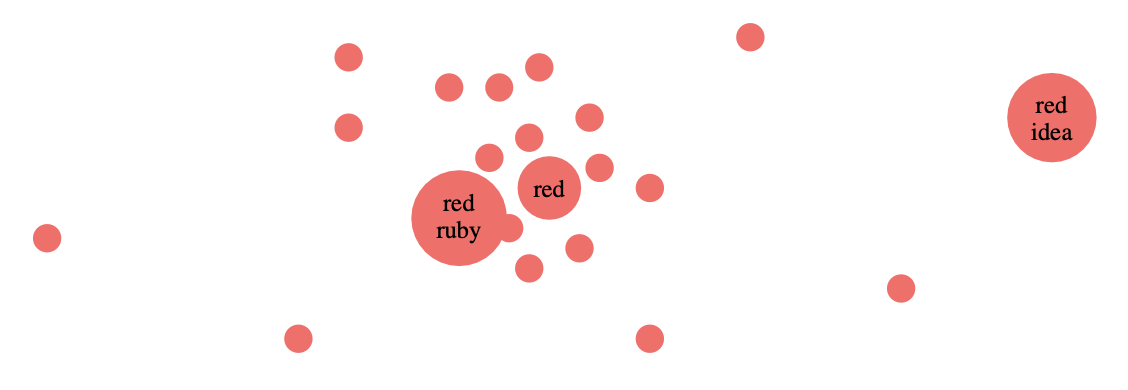In this way we can turn any language, or a collection of texts in a given language, into what Lawvere called a ‘generalized metric space’. It looks as if we are progressing slowly in our, probably futile, attempt to understand Alain Connes’ and Patrick Gauthier-Lafaye’s claim that ‘the unconscious is structured like a topos’. Even if we accept the fact that we can start from a collection of notes, there are a number of changes we need to make to the above approach: • there will be contextual links between these notes • we only want to retain the relevant snippets, not all of them • between these ‘highlights’ there may also be contextual links • texts can be related without having to be concatenations • we need to implement changes when new notes are added • … (much more) Perhaps, we should try to work on a specific ‘case’, and explore all technical tools that may help us to make progress. (tbc) Previously in this series: Next: Loading a second brain Last May, the meeting Lacan et Grothendieck, l’impossible rencontre? took place in Paris (see this post). Video’s of that meeting are now available online. Here’s the talk by Alain Connes and Patrick Gauthier-Lafaye on their book A l’ombre de Grothendieck et de Lacan : un topos sur l’inconscient ? (see this post ). Let’s quickly recall their main ideas: 1. The unconscious is structured as a topos (Jacques Lacan argued it was structured as a language), because we need a framework allowing logic without the law of the excluded middle for Lacan’s formulas of sexuation to make some sense at all. 2. This topos may differs from person to person, so we do not all share the same rules of logic (as observed in real life). 3. Consciousness is related to the points of the topos (they are not precise on this, neither in the talk, nor the book). 4. All these individual toposes are ruled by a classifying topos, and they see Lacan’s work as the very first steps towards trying to describe the unconscious by a geometrical theory (though his formulas are not first order). Surely these are intriguing ideas, if only we would know how to construct the topos of someone’s unconscious. Let’s go looking for clues. At the same meeting, there was a talk by Daniel Sibony: “Mathématiques et inconscient” Sibony started out as mathematician, then turned to psychiatry in the early 70ties. He was acquainted with both Grothendieck and Lacan, and even brought them together once, over lunch, some day in 1973. He makes a one-line appearance in Grothendieck’s Récoltes et Semailles, when G discribes his friends in ‘Survivre et Vivre’: “Daniel Sibony (who stayed away from this group, while pursuing its evolution out of the corner of a semi-disdainful, smirking eye)” In his talk, Sibony said he had a similar idea, 50 years before Connes and Gauthier-Lafaye (3.04 into the clip): “At the same time (early 70ties) I did a seminar in Vincennes, where I was a math professor, on the topology of dreams. At the time I didn’t have categories at my disposal, but I used fibered spaces instead. I showed how we could interpret dreams with a fibered space. This is consistent with the Freudian idea, except that Freud says we should take the list of words from the story of the dream and look for associations. For me, these associations were in the fibers, and these thoughts on fibers and sheaves have always followed me. And now, after 50 years I find this pretty book by Alain Connes and Patrick Gauthier-Lafaye on toposes, and see that my thoughts on dreams as sheaves and fibered spaces are but a special case of theirs.” This looks interesting. After all, Freud called dream interpretation the ‘royal road’ to the unconscious. “It is the ‘King’s highway’ along which everyone can travel to discover the truth of unconscious processes for themselves.” Sibony clarifies his idea in the interview L’utilisation des rêves en psychothérapie with Maryse Siksou. “The dream brings blocks of words, of “compacted” meanings, and we question, according to the good old method, each of these blocks, each of these points and which we associate around (we “unblock” around…), we let each point unfold according to the “fiber” which is its own. I introduced this notion of the dream as fibered space in an article in the review Scilicet in 1972, and in a seminar that I gave at the University of Vincennes in 1973 under the title “Topologie et interpretation des rêves”, to which Jacques Lacan and his close retinue attended throughout the year. The idea is that the dream is a sheaf, a bundle of fibers, each of which is associated with a “word” of the dream; interpretation makes the fibers appear, and one can pick an element from each, which is of course “displaced” in relation to the word that “produced” the fiber, and these elements are articulated with other elements taken in other fibers, to finally create a message which, once again, does not necessarily say the meaning of the dream because a dream has as many meanings as recipients to whom it is told, but which produces a strong statement, a relevant statement, which can restart the work.”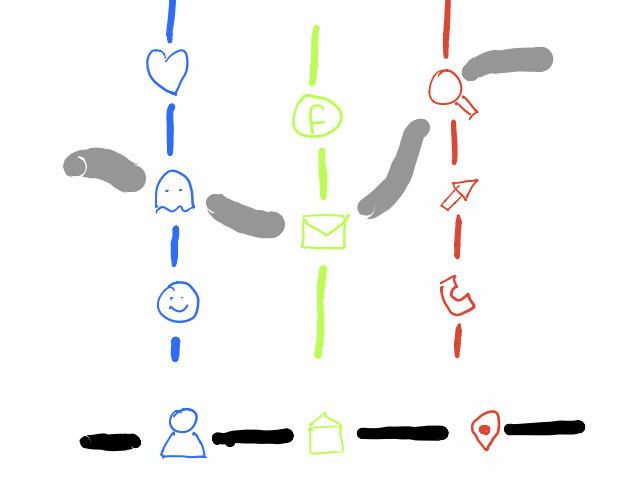Key images in the dream (the ‘points’ of the base-space) can stand for entirely different situations in someone’s life (the points in the ‘fiber’ over an image). The therapist’s job is to find a suitable ‘section’ in this ‘sheaf’ to further the theraphy. It’s a bit like translating a sentence from one language to another. Every word (point of the base-space) can have several possible translations with subtle differences (the points in the fiber over the word). It’s the translator’s job to find the best ‘section’ in this sheaf of possibilities. This translation-analogy is used by Daniel Sibony in his paper Traduire la passe: “It therefore operates just like the dream through articulated choices, from one fiber to another, in a bundle of speaking fibers; it articulates them by seeking the optimal section. In fact, the translation takes place between two fiber bundles, each in a language, but in the starting bundle the choice seems fixed by the initial text. However, more or less consciously, the translator “bursts” each word into a larger fiber, he therefore has a bundle of fibers where the given text seems after the fact a singular choice, which will produce another choice in the bundle of the other language.” This paper also contains a pre-ChatGPT story (we’re in 1998), in which the language model fails because it has far too few alternatives in its fibers: I felt it during a “humor festival” where I was approached by someone (who seemed to have some humor) and who was a robot. We had a brief conversation, very acceptable, beyond the conventional witticisms and knowing sighs he uttered from time to time to complain about the lack of atmosphere, repeating that after all we are not robots. I thought at first that it must be a walking walkie-talkie and that in fact I was talking to a guy who was remote control from his cabin. But the object was programmed; the unforeseen effects of meaning were all the more striking. To my question: “Who created you?” he answered with a strange word, a kind of technical god. I went on to ask him who he thought created me; his answer was immediate: “Oedipus”. (He knew, having questioned me, that I was a psychoanalyst.) The piquancy of his answer pleased me (without Oedipus, at least on a first level, no analyst). These bursts of meaning that we know in children, psychotics, to whom we attribute divinatory gifts — when they only exist, save their skin, questioning us about our being to defend theirs — , these random strokes of meaning shed light on the classic aftermaths where when a tile arrives, we hook it up to other tiles from the past, it ties up the pain by chaining the meaning. Anyway, the conversation continuing, the robot asked me to psychoanalyse him; I asked him what he was suffering from. His answer was immediate: “Oedipus”. Disappointing and enlightening: it shows that with each “word” of the interlocutor, the robot makes correspond a signifying constellation, a fiber of elements; choosing a word in each fiber, he then articulates the whole with obvious sequence constraints: a bit of readability and a certain phrasal push that leaves open the game of exchange. And now, in the fiber concerning the “psy” field, chance or constraint had fixed him on the same word, “Oedipus”, which, by repeating itself, closed the scene heavily. Okay, we have a first potential approximation to Connes and Gauthier-Lafaye’s elusive topos, a sheaf of possible interpretation of base-words in a language. But, the base-space is still rather discrete, or at best linearly ordered. And also in the fibers, and among the sections, there’s not much of a topology at work. Perhaps, we should have a look at applications of topology and/or topos theory in large language models? (tbc) Next: The shape of languages Last time, we’ve viewed major and minor triads (chords) as inscribed triangles in a regular$12$-gon.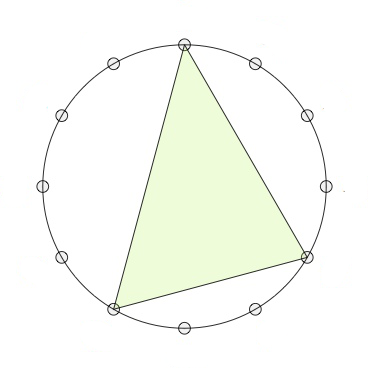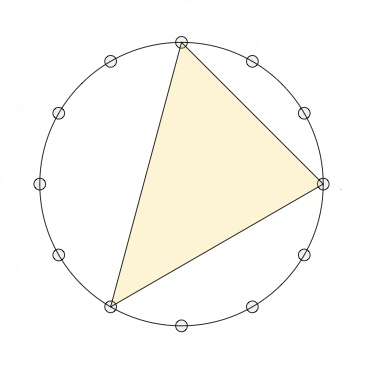If we move clockwise along the$12$-gon, starting from the endpoint of the longest edge (the root of the chord, here the$0$-vertex) the edges skip$3,2$and$4$vertices (for a major chord, here on the left the major$0$-chord) or$2,3$and$4$vertices (for a minor chord, here on the right the minor$0$-chord). The symmetries of the$12$-gon, the dihedral group$D_{12}$, act on the$24$major- and minor-chords transitively, preserving the type for rotations, and interchanging majors with minors for reflections. Mathematical Music Theoreticians (MaMuTh-ers for short) call this the$T/I$-group, and view the rotations of the$12$-gon as transpositions$T_k : x \mapsto x+k~\text{mod}~12$, and the reflections as involutions$I_k : x \mapsto -x+k~\text{mod}~12$. Note that the elements of the$T/I$-group act on the vertices of the$12$-gon, from which the action on the chord-triangles follows. There is another action on the$24$major and minor chords, mapping a chord-triangle to its image under a reflection in one of its three sides. Note that in this case the reflection$I_k$used will depend on the root of the chord, so this action on the chords does not come from an action on the vertices of the$12$-gon. There are three such operations: (pictures are taken from Alexandre Popoff’s blog, with the ‘funny names’ removed) The$P$-operation is reflection in the longest side of the chord-triangle. As the longest side is preserved,$P$interchanges the major and minor chord with the same root.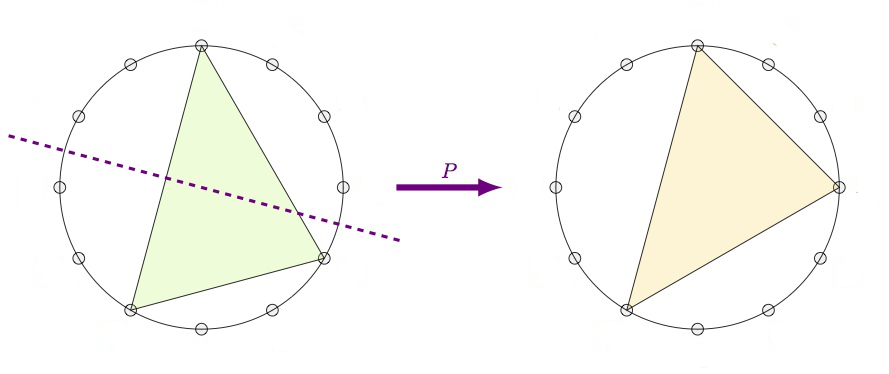The$L$-operation is refection in the shortest side. This operation interchanges a major$k$-chord with a minor$k+4~\text{mod}~12$-chord.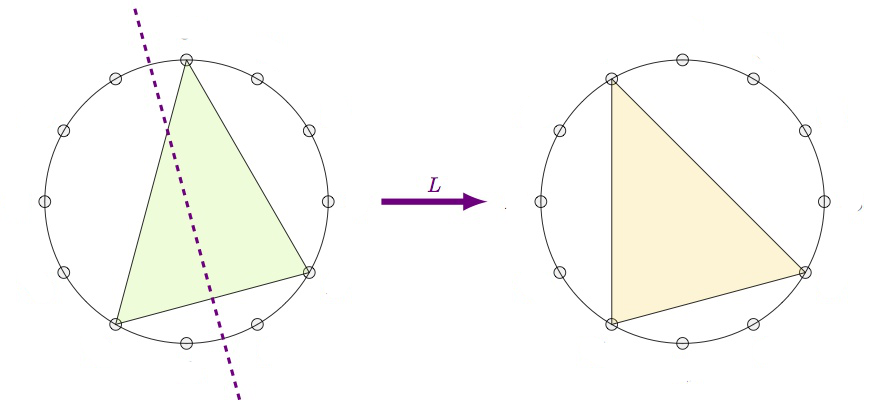Finally, the$R$-operation is reflection in the middle side. This operation interchanges a major$k$-chord with a minor$k+9~\text{mod}~12$-chord.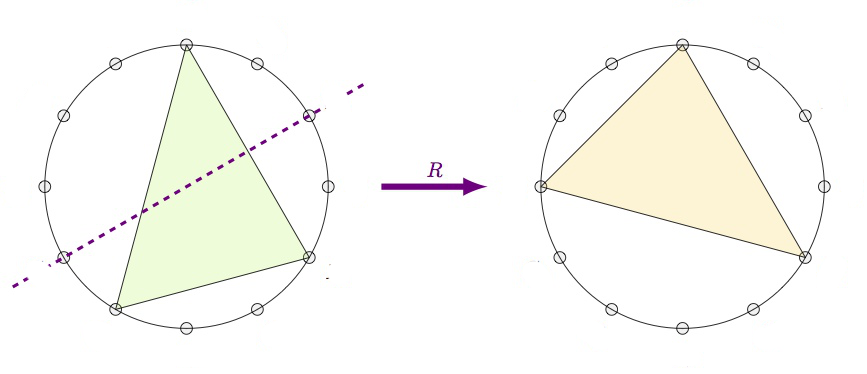From this it is already clear that the group generated by$P$,$L$and$R$acts transitively on the$24$major and minor chords, but what is this$PLR$-group? If we label the major chords by their root-vertex$1,2,\dots,12$(GAP doesn’t like zeroes), and the corresponding minor chords$13,14,\dots,24$, then these operations give these permutations on the$24$chords:  P:=(1,13)(2,14)(3,15)(4,16)(5,17)(6,18)(7,19)(8,20)(9,21)(10,22)(11,23)(12,24) L:=(1,17)(2,18)(3,19)(4,20)(5,21)(6,22)(7,23)(8,24)(9,13)(10,14)(11,15)(12,16) R:=(1,22)(2,23)(3,24)(4,13)(5,14)(6,15)(7,16)(8,17)(9,18)(10,19)(11,20)(12,21)  Then GAP gives us that the$PLR$-group is again isomorphic to$D_{12}$:  gap> G:=Group(P,L,R);; gap> Size(G); 24 gap> IsDihedralGroup(G); true  In fact, if we view both the$T/I$-group and the$PLR$-group as subgroups of the symmetric group$Sym(24)$via their actions on the$24$major and minor chords, these groups are each other centralizers! That is, the$T/I$-group and$PLR$-group are dual to each other. For more on this, there’s a beautiful paper by Alissa Crans, Thomas Fiore and Ramon Satyendra: Musical Actions of Dihedral Groups. What does this new MaMuTh info learns us more about our Elephant, the Topos of Triads, studied by Thomas Noll? Last time we’ve seen the eight element triadic monoid$T$of all affine maps preserving the three tones$\{ 0,4,7 \}$of the major$0$-chord, computed the subobject classified$\Omega$of the corresponding topos of presheaves, and determined all its six Grothendieck topologies, among which were these three: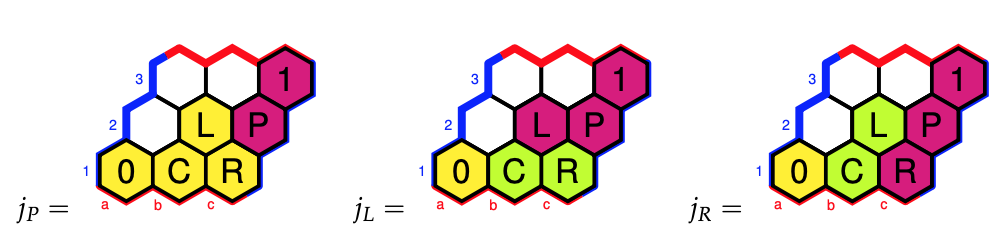Why did we label these Grothendieck topologies (and corresponding elements of$\Omega$) by$P$,$L$and$R$? We’ve seen that the sheafification of the presheaf$\{ 0,4,7 \}$in the triadic topos under the Grothendieck topology$j_P$gave us the sheaf$\{ 0,3,4,7 \}$, and these are the tones of the major$0$-chord together with those of the minor$0$-chord, that is the two chords in the$\langle P \rangle$-orbit of the major$0$-chord. The group$\langle P \rangle$is the cyclic group$C_2$. For the sheafication with respect to$j_L$we found the$T$-set$\{ 0,3,4,7,8,11 \}$which are the tones of the major and minor$0$-,$4$-, and$8$-chords. Again, these are exactly the six chords in the$\langle P,L \rangle$-orbit of the major$0$-chord. The group$\langle P,L \rangle$is isomorphic to$Sym(3)$. The$j_R$-topology gave us the$T$-set$\{ 0,1,3,4,6,7,9,10 \}$which are the tones of the major and minor$0$-,$3$-,$6$-, and$9$-chords, and lo and behold, these are the eight chords in the$\langle P,R \rangle$-orbit of the major$0$-chord. The group$\langle P,R \rangle$is the dihedral group$D_4$. More on this can be found in the paper Commuting Groups and the Topos of Triads by Thomas Fiore and Thomas Noll. The operations$P$,$L$and$R$on major and minor chords are reflexions in one side of the chord-triangle, so they preserve two of the three tones. There’s a distinction between the$P$and$L$operations and$R$when it comes to how the third tone changes. Under$P$and$L$the third tone changes by one halftone (because the corresponding sides skip an even number of vertices), whereas under$R$the third tone changes by two halftones (a full tone), see the pictures above. The$\langle P,L \rangle = Sym(3)$subgroup divides the$24$chords in four orbits of six chords each, three major chords and their corresponding minor chords. These orbits consist of the •$0$-,$4$-, and$8$-chords (see before) •$1$-,$5$-, and$9$-chords •$2$-,$6$-, and$10$-chords •$3$-,$7$-, and$11$-chords and we can view each of these orbits as a cycle tracing six of the eight vertices of a cube with one pair of antipodal points removed. These four ‘almost’ cubes are the NE-, SE-, SW-, and NW-regions of the Cube Dance Graph, from the paper Parsimonious Graphs by Jack Douthett and Peter Steinbach.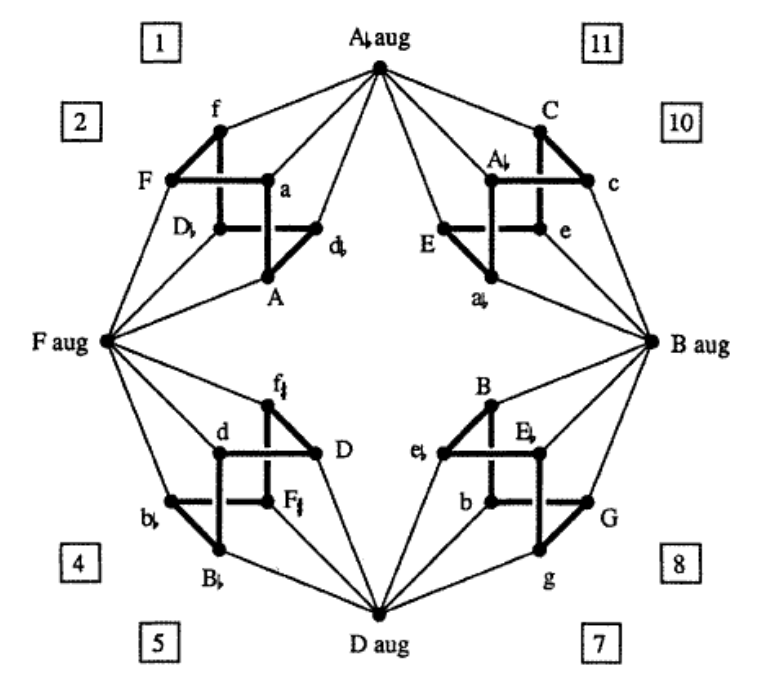To translate the funny names to our numbers, use this dictionary (major chords are given by a capital letter):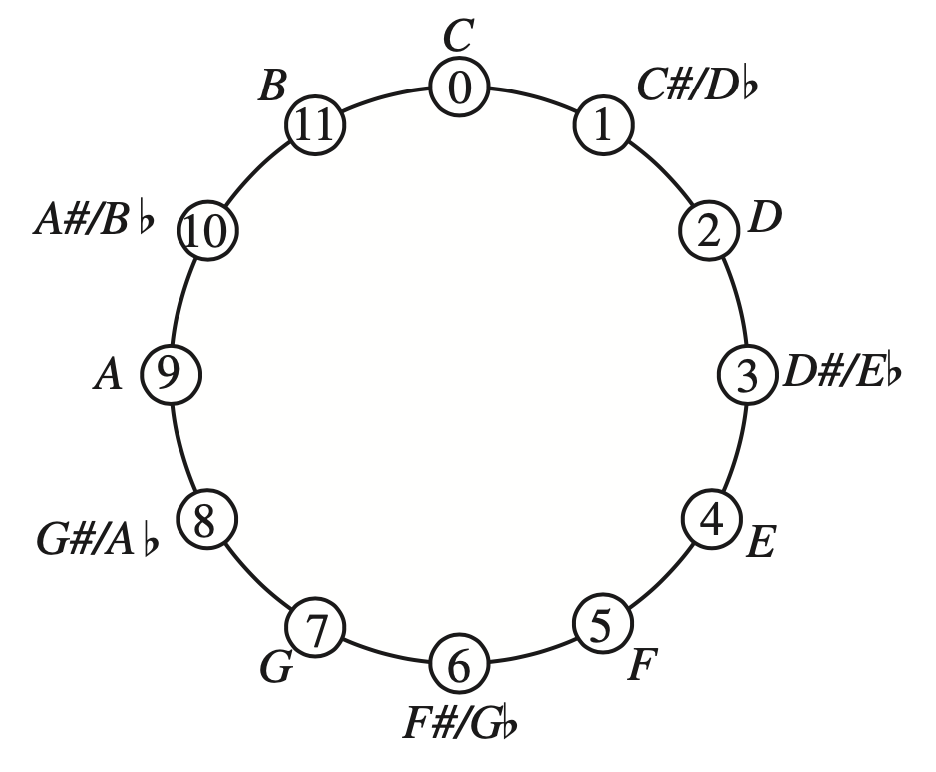The four extra chords (at the N, E, S, and P places) are augmented triads. They correspond to the triads$(0,4,8),~(1,5,9),~(2,6,10)$and$(3,7,11)$. That is, two triads are connected by an edge in the Cube Dance graph if they share two tones and differ by an halftone in the third tone. This graph screams for a group or monoid acting on it. Some of the edges we’ve already identified as the action of$P$and$L$on the$24$major and minor triads. Because the triangle of an augmented triad is equilateral, we see that they are preserved under$P$and$L$. But what about the edges connecting the regular triads to the augmented ones? If we view each edge as two directed arrows assigned to the same operation, we cannot do this with a transformation because the operation sends each augmented triad to six regular triads. Alexandre Popoff, Moreno Andreatta and Andree Ehresmann suggest in their paper Relational poly-Klumpenhouwer networks for transformational and voice-leading analysis that one might use a monoid generated by relations, and they show that there is such a monoid with$40$elements acting on the Cube Dance graph. Popoff claims that usual presheaf toposes, that is contravariant functors to$\mathbf{Sets}$are not enough to study transformational music theory. He suggest to use instead functors to$\mathbf{Rel}$, that is Sets with as the morphisms binary relations, and their compositions. Another Elephant enters the room… (to be continued) These three ideas (re)surfaced over the last two decades, claiming to have potential applications to major open problems: • (2000)$\mathbb{F}_1$-geometry tries to view$\mathbf{Spec}(\mathbb{Z})$as a curve over the field with one element, and mimic Weil’s proof of RH for curves over finite fields to prove the Riemann hypothesis. • (2012) IUTT, for Inter Universal Teichmuller Theory, the machinery behind Mochizuki’s claimed proof of the ABC-conjecture. • (2014) topos theory : Connes and Consani redirected their RH-attack using arithmetic sites, while Lafforgue advocated the use of Caramello’s bridges for unification, in particular the Langlands programme. It is difficult to voice an opinion about the (presumed) current state of such projects without being accused of being either a believer or a skeptic, resorting to group-think or being overly critical. We lack the vocabulary to talk about the different phases a mathematical idea might be in. Such a vocabulary exists in (information) technology, the five phases of the Gartner hype cycle to represent the maturity, adoption, and social application of a certain technology : 1. Technology Trigger 2. Peak of Inflated Expectations 3. Trough of Disillusionment 4. Slope of Enlightenment 5. Plateau of ProductivityThis model can then be used to gauge in which phase several emerging technologies are, and to estimate the time it will take them to reach the stable plateau of productivity. Here’s Gartner’s recent Hype Cycle for emerging Artificial Intelligence technologies.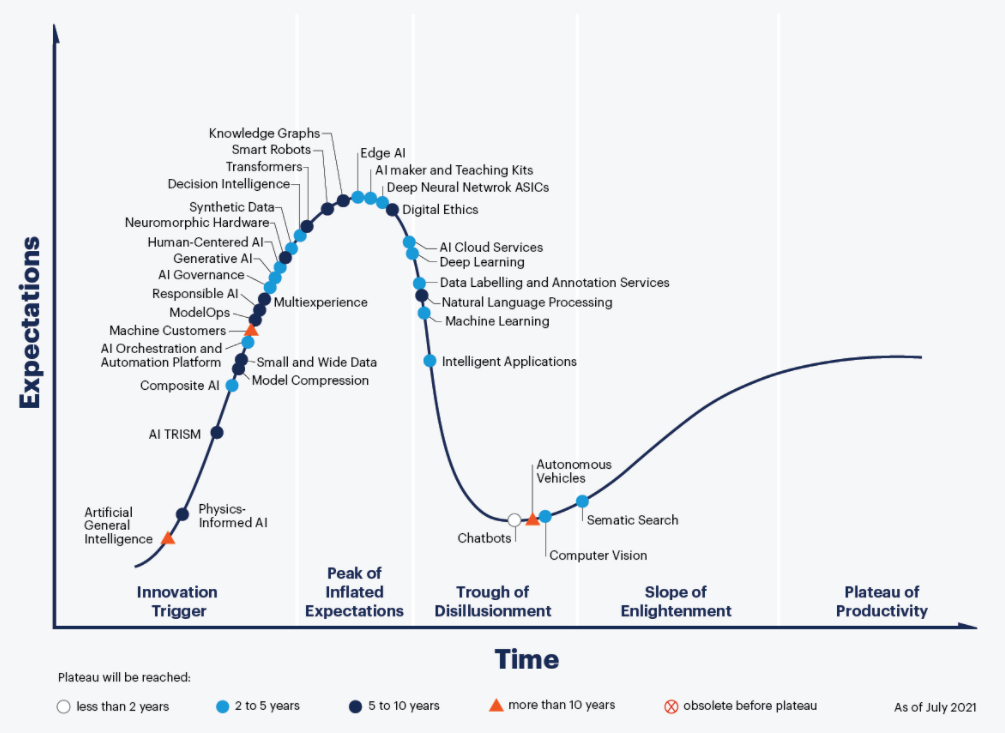Picture from Gartner Hype Cycle for AI 2021 What might these phases be in the hype cycle of a mathematical idea? 1. Technology Trigger: a new idea or analogy is dreamed up, marketed to be the new approach to that problem. A small group of enthusiasts embraces the idea, and tries to supply proper definitions and the very first results. 2. Peak of Inflated Expectations: the idea spreads via talks, blogposts, mathoverflow and twitter, and now has enough visibility to justify the first conferences devoted to it. However, all this activity does not result in major breakthroughs and doubt creeps in. 3. Trough of Disillusionment: the project ran out of steam. It becomes clear that existing theories will not lead to a solution of the motivating problem. Attempts by key people to keep the idea alive (by lengthy papers, regular meetings or seminars) no longer attract new people to the field. 4. Slope of Enlightenment: the optimistic scenario. One abandons the original aim, ditches the myriad of theories leading nowhere, regroups and focusses on the better ideas the project delivered. A negative scenario is equally possible. Apart for a few die-hards the idea is abandoned, and on its way to the graveyard of forgotten ideas. 5. Plateau of Productivity: the polished surviving theory has applications in other branches and becomes a solid tool in mathematics. It would be fun so see more knowledgable people draw such a hype cycle graph for recent trends in mathematics. Here’s my own (feeble) attempt to gauge where the three ideas mentioned at the start are in their cycles, and here’s why: • IUTT: recent work of Kirti Joshi, for example this, and this, and that, draws from IUTT while using conventional language and not making exaggerated claims. •$\mathbb{F}_1$: the preliminary programme of their seminar shows little evidence the$\mathbb{F}_1$-community learned from the past 20 years. • Topos: Developing more general theory is not the way ahead, but concrete examples may carry surprises, even though Gabriel’s topos will remain elusive.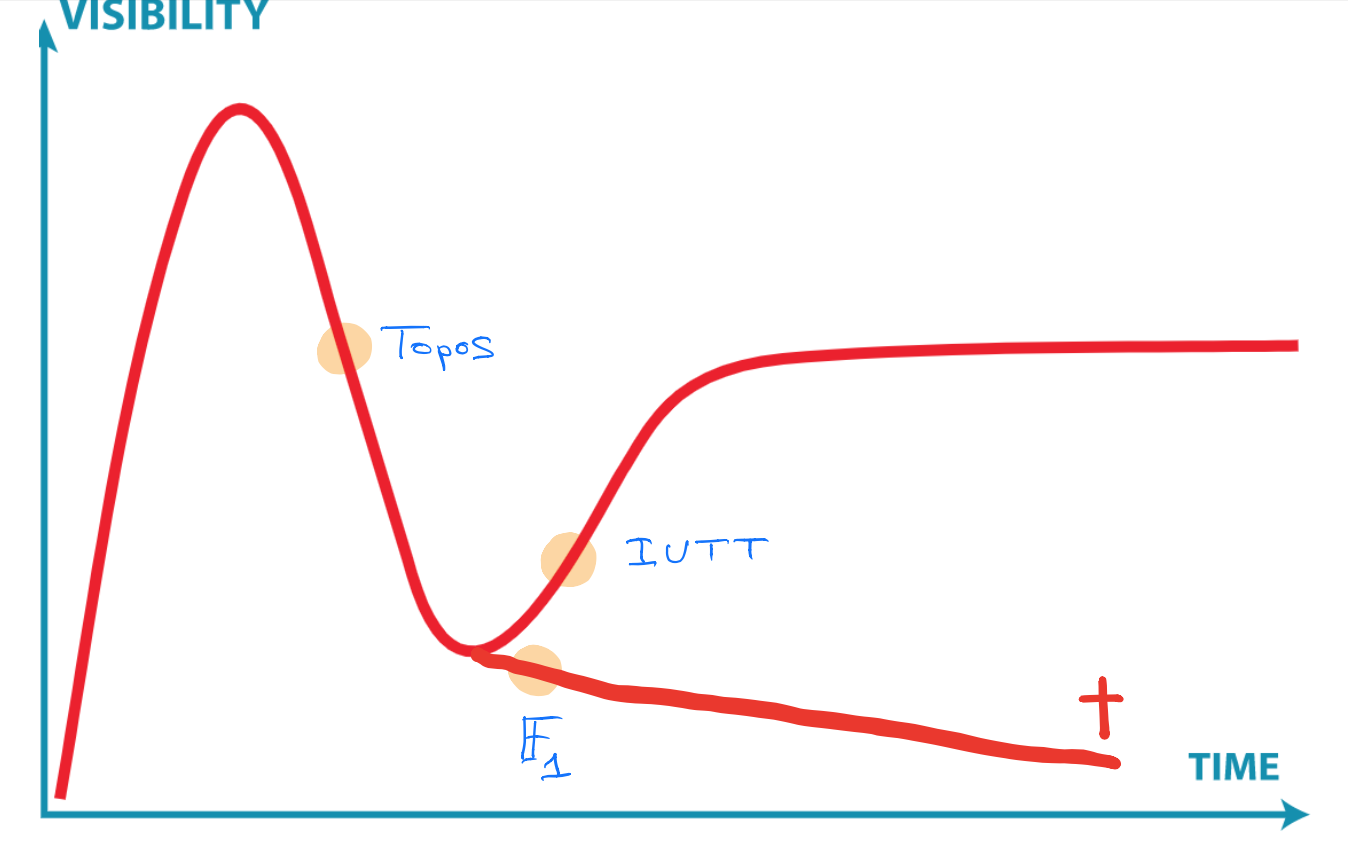Clearly, you don’t agree, and that’s fine. We now have a common terminology, and you can point me to results or events I must have missed, forcing me to redraw my graph. Brendan Fong, David Spivak and Remy Tuyeras cooked up a vast generalisation of neural networks in their paper Backprop as Functor: A compositional perspective on supervised learning. Here’s a nice introduction to neural networks for category theorists by Bruno Gavranovic. At 1.49m he tries to explain supervised learning with neural networks in one slide. Learners show up later in the talk.$\mathbf{Poly}$is the category of all polynomial functors, that is, things of the form $p = \sum_{i \in p(1)} y^{p[i]}~:~\mathbf{Sets} \rightarrow \mathbf{Sets} \qquad S \mapsto \bigsqcup_{i \in p(1)} Maps(p[i],S)$ with$p(1)$and all$p[i]$sets. Last time I gave Spivak’s ‘corolla’ picture to think about such functors. I prefer to view$p \in \mathbf{Poly}$as an horribly discrete ‘sheaf’$\mathcal{P}$over the ‘space’$p(1)$with stalk$p[i]=\mathcal{P}_i$at point$i \in p(1)$.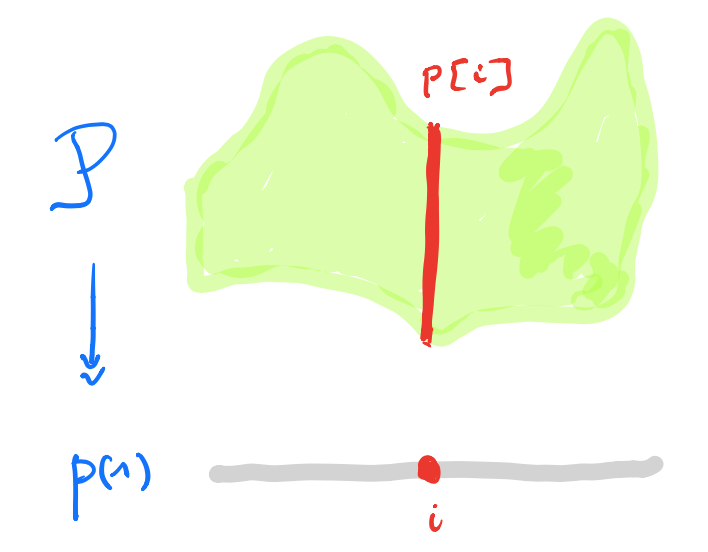A morphism$p \rightarrow q$in$\mathbf{Poly}$is a map$\varphi_1 : p(1) \rightarrow q(1)$, together with for all$i \in p(1)$a map$\varphi^{\#}_i : q[\varphi_1(i)] \rightarrow p[i]$. In the sheaf picture, this gives a map of sheaves over the space$p(1)$from the inverse image sheaf$\varphi_1^* \mathcal{Q}$to$\mathcal{P}$.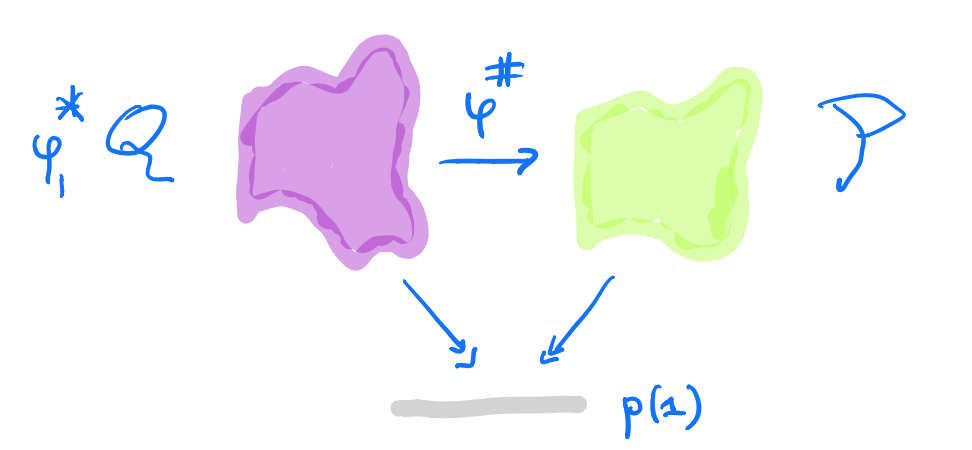But, unless you dream of sheaves in the night, by all means stick to Spivak’s corolla picture. A learner$A \rightarrow B$between two sets$A$and$B$is a complicated tuple of things$(P,I,U,R)$: •$P$is a set, a parameter space of some maps from$A$to$B$. •$I$is the interpretation map$I : P \times A \rightarrow B$describing the maps in$P$. •$U$is the update map$U : P \times A \times B \rightarrow P$, the learning procedure. The idea is that$U(p,a,b)$is a map which sends$a$closer to$b$than the map$p$did. •$R$is the request map$R : P \times A \times B \rightarrow A$. Here’s a nice application of$\mathbf{Poly}$’s set-up: Morphisms$\mathbf{P y^P \rightarrow Maps(A,B) \times Maps(A \times B,A) y^{A \times B}}$in$\mathbf{Poly}$coincide with learners$\mathbf{A \rightarrow B}$with parameter space$\mathbf{P}$. This follows from unpacking the definition of morphism in$\mathbf{Poly}$and the process CT-ers prefer to call Currying. The space-map$\varphi_1 : P \rightarrow Maps(A,B) \times Maps(A \times B,A)$gives us the interpretation and request-map, whereas the sheaf-map$\varphi^{\#}$gives us the more mysterious update-map$P \times A \times B \rightarrow P$.$\mathbf{Learn(A,B)}$is the category with objects all the learners$A \rightarrow B$(for all paramater-sets$P$), and with morphisms defined naturally, that is, maps between the parameter-sets, compatible with the structural maps. A surprising result from David Spivak’s paper Learners’ Languages is$\mathbf{Learn(A,B)}$is a topos. In fact, it is the topos of all set-valued representations of a (huge) directed graph$\mathbf{G_{AB}}$. This will take some time. Let’s bring some dynamics in. Take any polynmial functor$p \in \mathbf{Poly}$and fix a morphism in$\mathbf{Poly}$$\varphi = (\varphi_1,\varphi[-])~:~p(1) y^{p(1)} \rightarrow p$ with space-map$\varphi_1$the identity map. We form a directed graph: • the vertices are the elements of$p(1)$, • vertex$i \in p(1)$is the source vertex of exactly one arrow for every$a \in p[i]$, • the target vertex of that arrow is the vertex$\phi[i](a) \in p(1)$. Here’s one possibility from Spivak’s paper for$p = 2y^2 + 1$, with the coefficient$2$-set$\{ \text{green dot, yellow dot} \}$, and with$1$the singleton$\{ \text{red dot} \}$.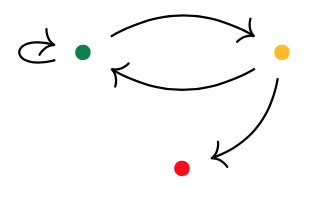Start at one vertex and move after a minute along a directed edge to the next (possibly the same) vertex. The potential evolutions in time will then form a tree, with each node given a label in$p(1)$. If we start at the green dot, we get this tree of potential time-evolutions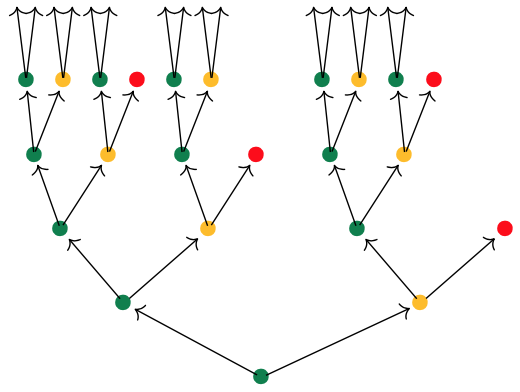There are exactly$\# p[i]$branches leaving a node labeled$i \in p(1)$, and all subtrees emanating from equal labelled nodes are isomorphic. If we had started at the yellow dot we had obtained a labelled tree isomorphic to the subtree emanating here from any yellow dot. We can do the same things for any morphism in$\mathbf{Poly}$of the form $\varphi = (\varphi_1,\varphi[-])~:~Sy^S \rightarrow p$ Now, we have a directed graph with vertices the elements$s \in S$, with as many edges leaving vertex$s$as there are elements$a \in p[\varphi_1(s)]$, and with the target vertex of the edge labeled$a$starting in$s$the vertex$\varphi[\varphi_1(s)](A)$. Once we have this directed graph on$\# S$vertices we can label vertex$s$with the label$\varphi_1(s)$from$p(1)$. In this way, the time evolutions starting at a vertex$s \in S$will give us a$p(1)$-labelled rooted tree. But now, it is possibly that two distinct vertices can have the same$p(1)$-labeled tree of evolutions. But also, trees corresponding to equal labeled vertices can be different. Right, I guess we’re ready to define the graph$G_{AB}$and prove that$\mathbf{Learn(A,B)}$is a topos. In the case of learners, we have the target polynomial functor$p=C y^{A \times B}$with$C = Maps(A,B) \times Maps(A \times B,A)$, that is $p(1) = C \quad \text{and all} \quad p[i]=A \times B$ Start with the free rooted tree$T$having exactly$\# A \times B$branches growing from each node. Here’s the directed graph$G_{AB}$: • vertices$v_{\chi}$correspond to the different$C$-labelings of$T$, one$C$-labeled rooted tree$T_{\chi}$for every map$\chi : vtx(T) \rightarrow C$, • arrows$v_{\chi} \rightarrow v_{\omega}$if and only if$T_{\omega}$is the rooted$C$-labelled tree isomorphic to the subtree of$T_{\chi}$rooted at one step from the root. A learner$\mathbf{A \rightarrow B}$gives a set-valued representation of$\mathbf{G_{AB}}$. We saw that a learner$A \rightarrow B$is the same thing as a morphism in$\mathbf{Poly}$$\varphi = (\varphi_1,\varphi[-])~:~P y^P \rightarrow C y^{A \times B}$ with$P$the parameter set of maps. Here’s what we have to do: 1. Draw the directed graph on vertices$p \in P$giving the dynamics of the morphism$\varphi$. This graph describes how the learner can cycle through the parameter-set. 2. Use the map$\varphi_1$to label the vertices with elements from$C$.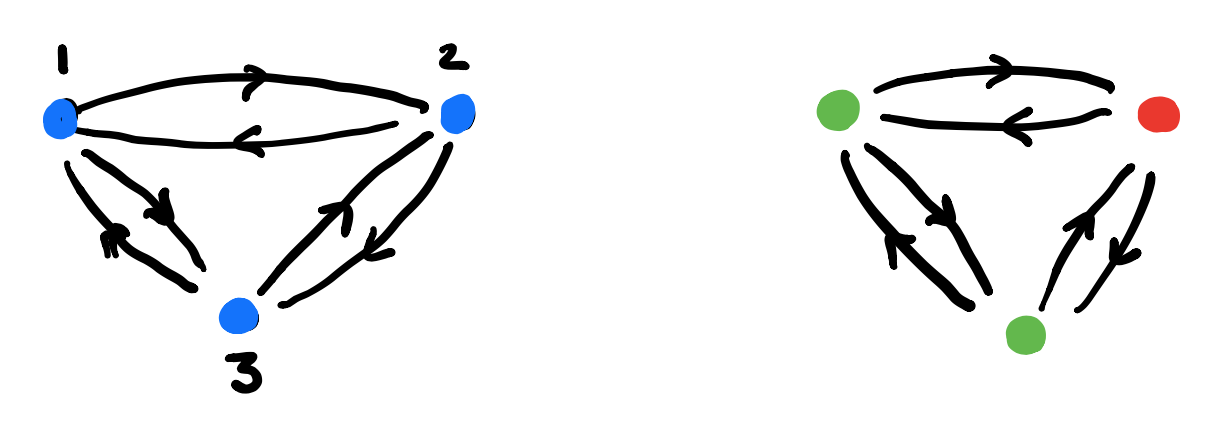3. For each vertex draw the rooted$C$-labeled tree of potential time-evolutions starting in that vertex. In this example the time-evolutions of the two green vertices are the same, but in general they can be different.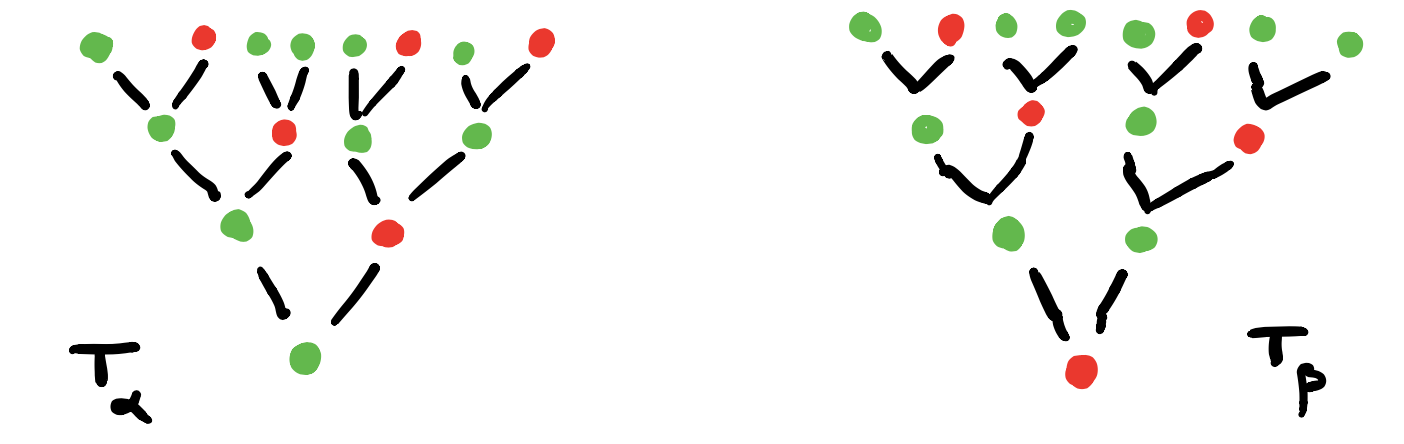4. Find the vertices in$G_{AB}$determined by these$C$-labeled trees and note that they span a full subgraph of$G_{AB}$.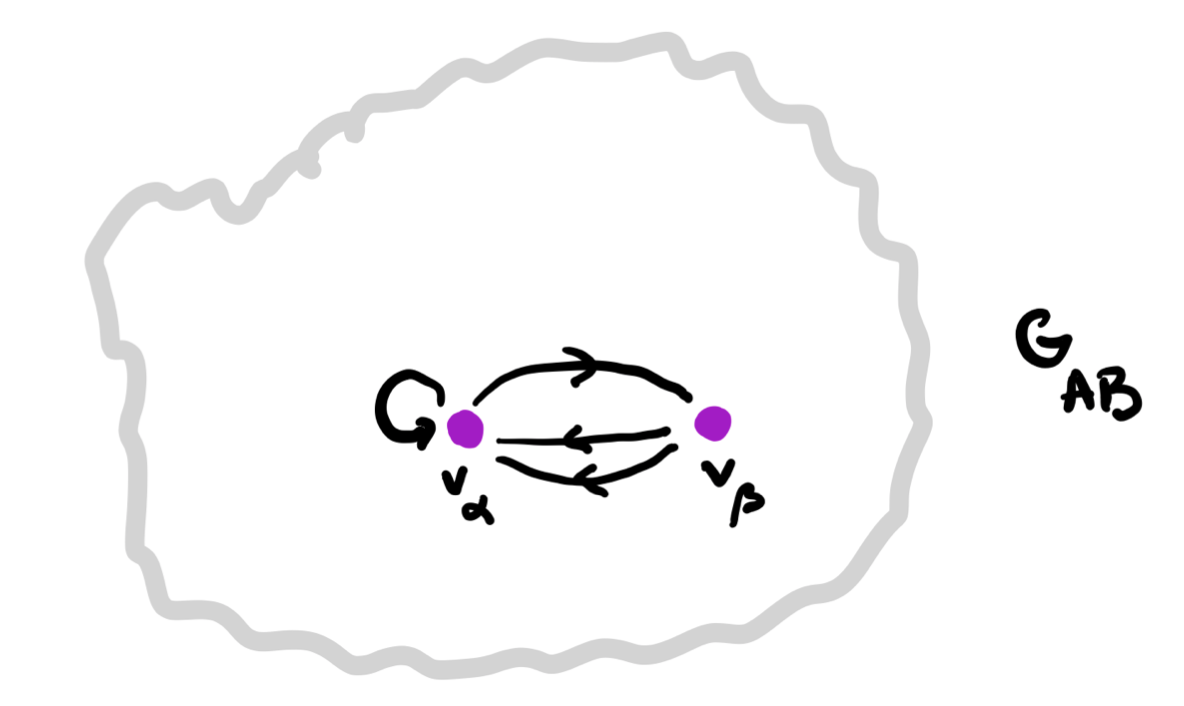5. The vertex-set$P_v$consists of all elements from$p$whose ($C$-labeled) vertex has evolution-tree$T_v$. If$v \rightarrow w$is a directed edge in$G_{AB}$corresponding to an element$(a,b) \in A \times B$, then the map on the vertex-sets corresponding to this edge is $f_{v,(a,b)}~:~P_v \rightarrow P_w \qquad p \mapsto \varphi[\varphi_1(p)](a,b)$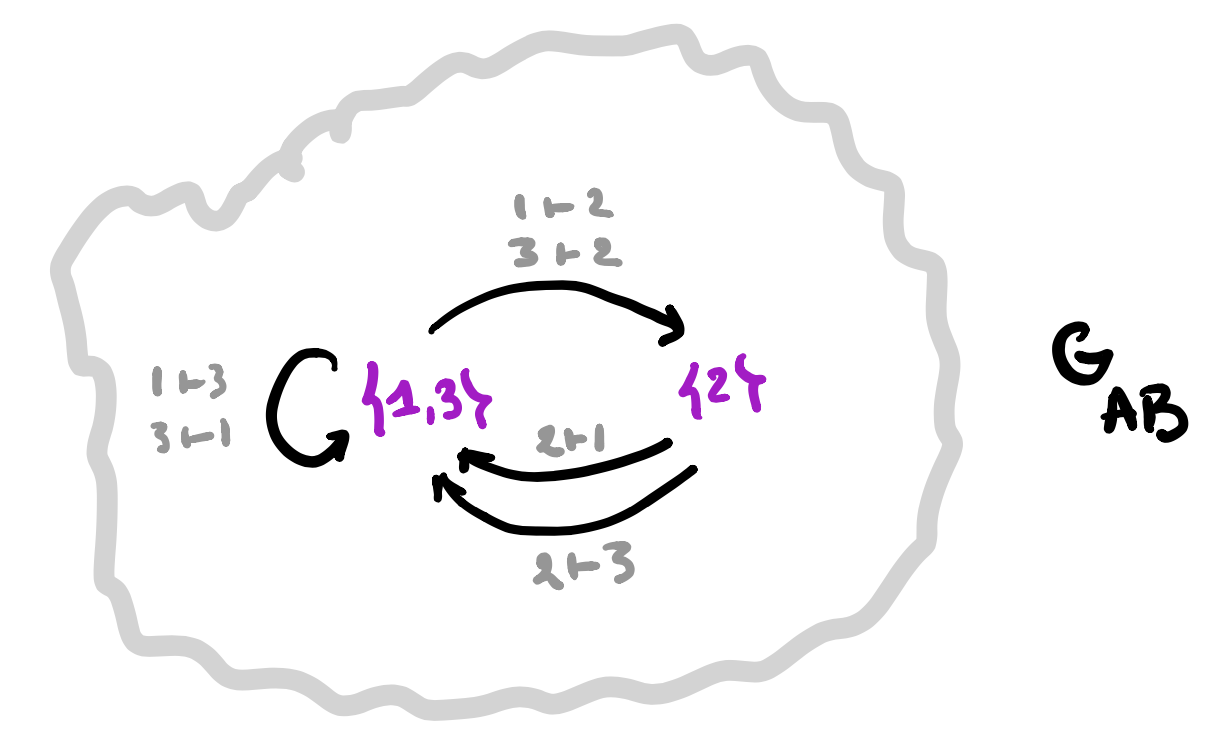A set-valued representation of$\mathbf{G_{AB}}$gives a learner$\mathbf{A \rightarrow B}$. 1. Take a set-valued representation of$G_{AB}$, that is, the finite or infinite collection of vertices$V$in$G_{AB}$where the vertex-set$P_v$is non-empty. Note that these vertices span a full subgraph of$G_{AB}$. And, for each directed arrow$v \rightarrow w$in this subgraph, labeled by an element$(a,b) \in A \times B$we have a map $f_{v,(a,b)}~:~P_v \rightarrow P_w$ 2. The parameter set of our learner will be$P = \sqcup_v P_v$, the disjoint union of the non-empty vertex-sets. 3. The space-map$\varphi_1 : P \rightarrow C$will send an element in$P_v$to the$C$-label of the root of the tree$T_v$. This gives us already the interpretation and request maps $I : P \times A \rightarrow B \quad \text{and} \quad R : P \times A \times B \rightarrow A$ 4. The update map$U : P \times A \times B \rightarrow P$follows from the sheaf-map we can define stalk-wise $\varphi[\varphi_1(p)](a,b) = f_{v,(a,b)}(p)$ if$p \in P_v$. That’s all folks!$\mathbf{Learn(A,B)}$is equivalent to the (covariant) functors$\mathbf{G_{AB} \rightarrow Sets}$. Changing the directions of all arrows in$G_{AB}$any covariant functor$\mathbf{G_{AB} \rightarrow Sets}$becomes a contravariant functor$\mathbf{G_{AB}^o \rightarrow Sets}$, making$\mathbf{Learn(A,B)}$an honest to Groth topos! Every topos comes with its own logic, so we have a ‘learners’ logic’. (to be continued) Some months ago, Peter Scholze wrote a guest post on the Xena-blog: Liquid tensor experiment, proposing a challenge to formalise the proof of one of his results with Dustin Clausen on condensed mathematics. Scholze and Clausen ran a masterclass in Copenhagen on condensed mathematics, which you can binge watch on YouTube starting here Scholze also gave two courses on the material in Bonn of which the notes are available here and here. Condensed mathematics claims that topological spaces are the wrong definition, and that one should replace them with the slightly different notion of condensed sets. So, let’s find out what a condensed set is. Definition: Condensed sets are sheaves (of sets) on the pro-étale site of a point. (there’s no danger we’ll have to rewrite our undergraduate topology courses just yet…) In his blogpost, Scholze motivates this paradigm shift by observing that the category of topological Abelian groups is not Abelian (if you put a finer topology on the same group then the identity map is not an isomorphism but doesn’t have a kernel nor cokernel) whereas the category of condensed Abelian groups is. It was another Clausen-Scholze result in the blogpost that caught my eye. But first, for something completely different. In “Musical creativity”, Guerino Mazzola and co-authors introduce a seven steps path to creativity.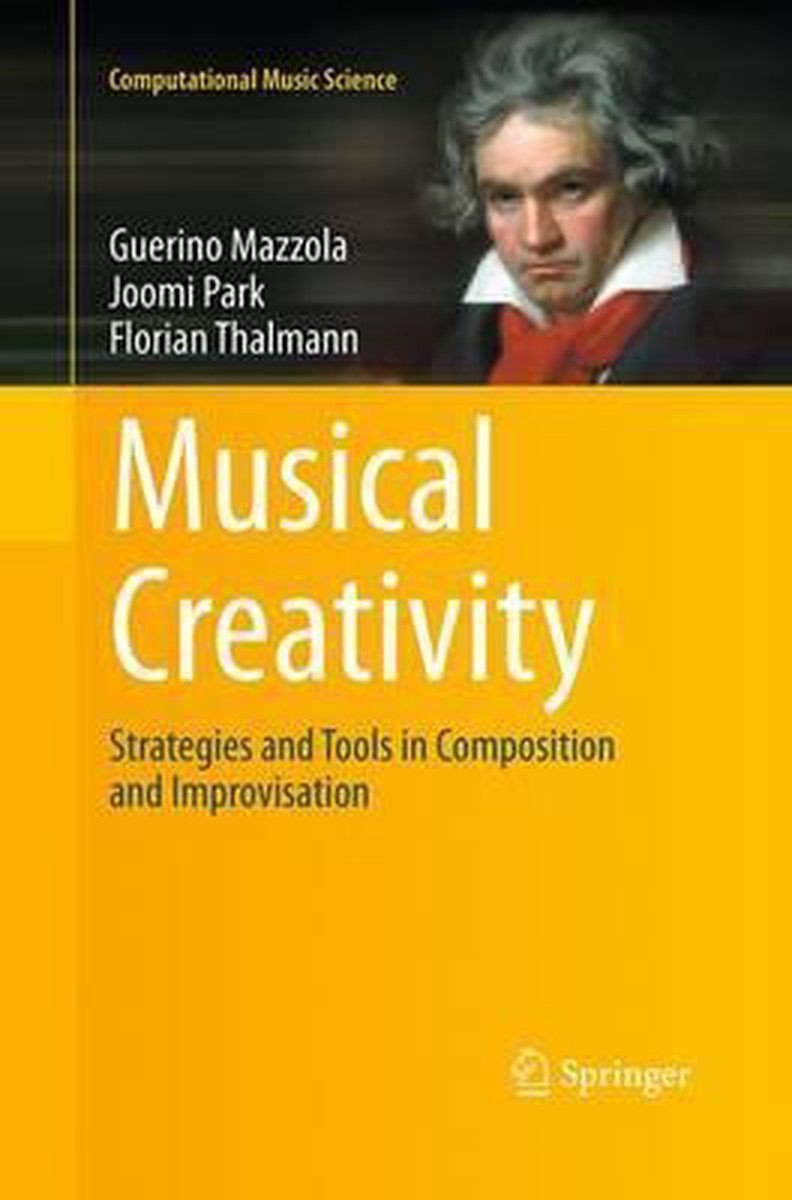Here they are: 1. Exhibiting the open question 2. Identifying the semiotic context 3. Finding the question’s critical sign 4. Identifying the concept’s walls 5. Opening the walls 6. Displaying extended wall perspectives 7. Evaluating the extended walls Looks like a recipe from distant flower-power pot-infused times, no? In Towards a Categorical Theory of Creativity for Music, Discourse, and Cognition, Mazzola, Andrée Ehresmann and co-authors relate these seven steps to the Yoneda lemma. 1. Exhibiting the open question = to understand the object$A$2. Identifying the semiotic context = to describe the category$\mathbf{C}$of which$A$is an object 3. Finding the question’s critical sign =$A$(?!) 4. Identifying the concept’s walls = the uncontrolled behaviour of the Yoneda functor $@A~:~\mathbf{C} \rightarrow \mathbf{Sets} \qquad C \mapsto Hom_{\mathbf{C}}(C,A)$ 5. Opening the walls = finding an objectively creative subcategory$\mathbf{A}$of$\mathbf{C}$6. Displaying extended wall perspectives = calculate the colimit$C$of a creative diagram 7. Evaluating the extended walls = try to understand$A$via the isomorphism$C \simeq A$. (Actually, I first read about these seven categorical steps in another paper which might put a smile on your face: The Yoneda path to the Buddhist monk blend.) Remains to know what a ‘creative’ subcategory is. The creative moment comes in here: could we not find a subcategory$\mathbf{A}$of$\mathbf{C}$such that the functor $Yon|_{\mathbf{A}}~:~\mathbf{C} \rightarrow \mathbf{PSh}(\mathbf{A}) \qquad A \mapsto @A|_{\mathbf{A}}$ is still fully faithful? We call such a subcategory creative, and it is a major task in category theory to find creative categories which are as small as possible. All the ingredients are here, but I had to read Peter Scholze’s blogpost before the penny dropped. Let’s try to view condensed sets as the result of a creative process. 1. Exhibiting the open question: you are a topologist and want to understand a particular compact Hausdorff space$X$. 2. Identifying the semiotic context: you are familiar with working in the category$\mathbf{Tops}$of all topological spaces with continuous maps as morphisms. 3. Finding the question’s critical sign: you want to know what differentiates your space$X$from all other topological spaces. 4. Identifying the concept’s walls: you can probe your space$X$with continuous maps from other topological spaces. That is, you can consider the contravariant functor (or presheaf on$\mathbf{Tops}$) $@X~:~\mathbf{Tops} \rightarrow \mathbf{Sets} \qquad Y \mapsto Cont(Y,X)$ and Yoneda tells you that this functor, up to equivalence, determines the space$X$upto homeomorphism. 5. Opening the walls: Tychonoff tells you that among all compact Hausdorff spaces there’s a class of pretty weird examples: inverse limits of finite sets (or a bit pompous: the pro-etale site of a point). These limits form a subcategory$\mathbf{ProF}$of$\mathbf{Tops}$. 6. Displaying extended wall perspectives: for every inverse limit$F \in \mathbf{ProF}$(for ‘pro-finite sets’) you can look at the set$\mathbf{X}(F)=Cont(F,X)$of all continuous maps from$F$to$X$(that is, all probes of$X$by$F$) and this functor $\mathbf{X}=@X|_{\mathbf{ProF}}~:~\mathbf{ProF} \rightarrow \mathbf{Sets} \qquad F \mapsto \mathbf{X}(F)$ is a sheaf on the pre-etale site of a point, that is,$\mathbf{X}$is the condensed set associated to$X$. 7. Evaluating the extended walls: Clausen and Scholze observe that the assignment$X \mapsto \mathbf{X}$embeds compact Hausdorff spaces fully faithful into condensed sets, so we can recover$X$up to homeomorphism as a colimit from the condenset set$\mathbf{X}$. Or, in Mazzola’s terminology:$\mathbf{ProF}$is a creative subcategory of$\mathbf{(cH)Tops}\$ (all compact Hausdorff spaces).

It would be nice if someone would come up with a new notion for me to understand Mazzola’s other opus “The topos of music” (now reprinted as a four volume series).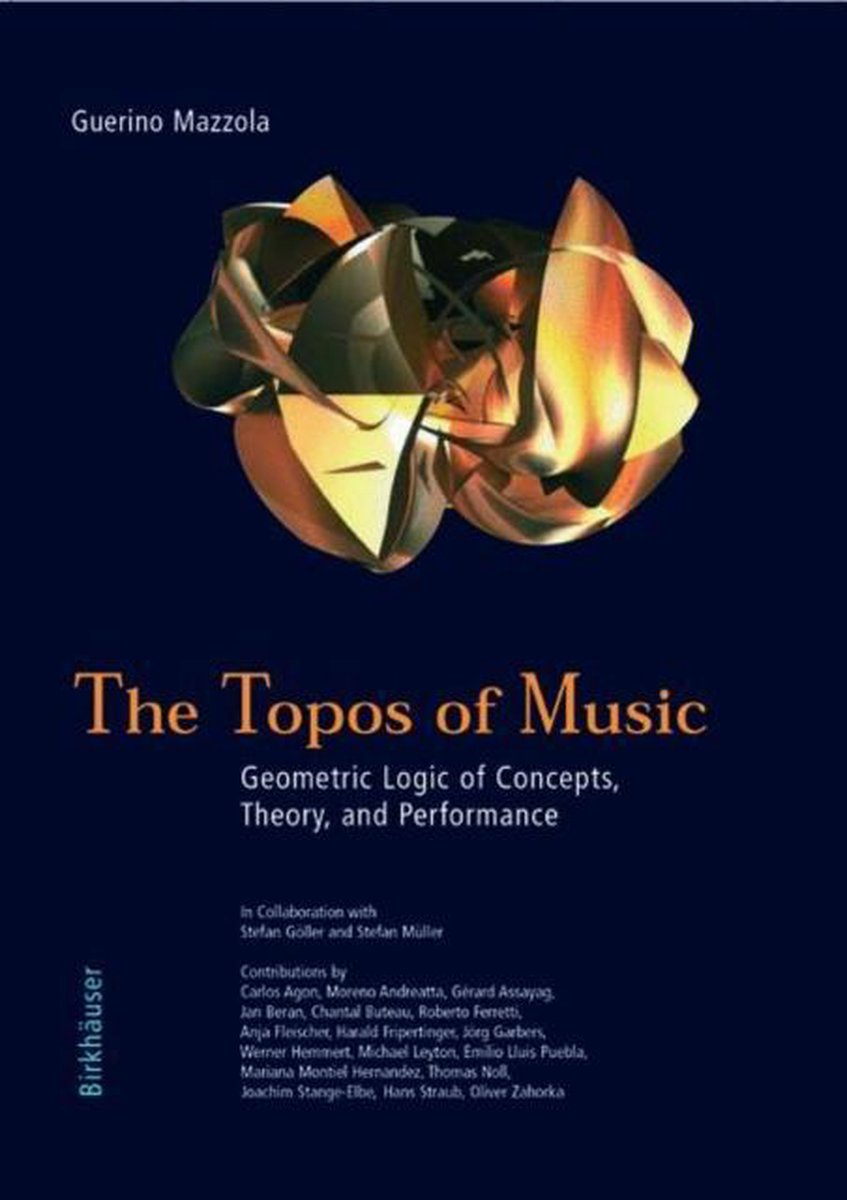No kidding, this is the final sentence of Le spectre d’Atacama, the second novel by Alain Connes (written with Danye Chéreau (IRL Mrs. AC) and his former Ph.D. advisor Jacques Dixmier).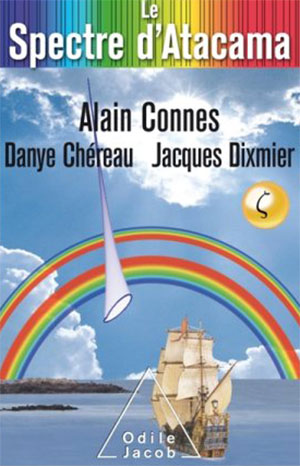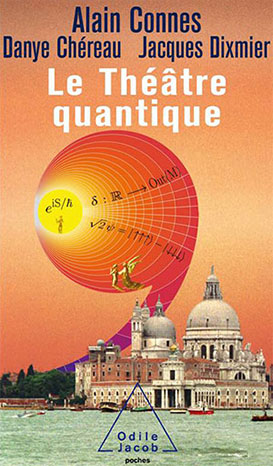The book has a promising start. Armand Lafforet (IRL AC) is summoned by his friend Rodrigo to the Chilean observatory Alma in the Altacama desert. They have observed a mysterious spectrum, and need his advice.

Armand drops everything and on the flight he lectures the lady sitting next to him on proofs by induction (breaking up chocolate bars), and recalls a recent stay at the La Trappe Abbey, where he had an encounter with (the ghost of) Alexander Grothendieck, who urged him to ‘Follow the motif!’.

“Comment était-il arrivé là? Il possédait surement quelques clés. Pourquoi pas celles des songes?” (How did he get
there? Surely he owned some keys, why not those of our dreams?)

A few pages further there’s this on the notion of topos (my attempt to translate):

“The notion of space plays a central role in mathematics. Traditionally we represent it as a set of points, together with a notion of neighborhood that we call a ‘topology’. The universe of these new spaces, ‘toposes’, unveiled by Grothendieck, is marvellous, not only for the infinite wealth of examples (it contains, apart from the ordinary topological spaces, also numerous instances of a more combinatorial nature) but because of the totally original way to perceive space: instead of appearing on the main stage from the start, it hides backstage and manifests itself as a ‘deus ex machina’, introducing a variability in the theory of sets.”

So far, so good.

We have a mystery, tidbits of mathematics, and allusions left there to put a smile on any Grothendieck-aficionado’s face.

But then, upon arrival, the story drops dead.

Rodrigo has been taken to hospital, and will remain incommunicado until well in the final quarter of the book.

As the remaining astronomers show little interest in Alain’s (sorry, Armand’s) first lecture, he decides to skip the second, and departs on a hike to the ocean. There, he takes a genuine sailing ship in true Jules Verne style to the lighthouse at he end of the world.

All this drags on for at least half a year in time, and two thirds of the book’s length. We are left in complete suspense when it comes to the mysterious Atacama spectrum.

Perhaps the three authors deliberately want to break with existing conventions of story telling?

I had a similar feeling when reading their first novel Le Theatre Quantique. Here they spend some effort to flesh out their heroine, Charlotte, in the first part of the book. But then, all of a sudden, their main character is replaced by a detective, and next by a computer.

Anyway, when Armand finally reappears at the IHES the story picks up pace.

The trio (Armand, his would-be-lover Charlotte, and Ali Ravi, Cern’s computer guru) convince CERN to sell its main computer to an American billionaire with the (fake) promise of developing a quantum computer. Incidentally, they somehow manage to do this using Charlotte’s history with that computer (for this, you have to read ‘Le Theatre Quantique’).

By their quantum-computing power (Shor and quantum-encryption pass the revue) they are able to decipher the Atacame spectrum (something to do with primes and zeroes of the zeta function), send coded messages using quantum entanglement, end up in the Oval Office and convince the president to send a message to the ‘Riemann sphere’ (another fun pun), and so on, and on.

The book ends with a twist of the classic tale of the mathematician willing to sell his soul to the devil for a (dis)proof of the Riemann hypothesis:

After spending some time in purgatory, the mathematician gets a meeting with God and asks her the question “Is the Riemann hypothesis true?”.

“Of course”, God says.

“But how can you know that all non-trivial zeroes of the zeta function have real part 1/2?”, Armand asks.

And God replies:

“Simple enough, I can see them all at once. But then, don’t forget I’m God. I can see the disappointment in your face, yes I can read in your heart that you are frustrated, that you desire an explanation…

Well, we’re going to fix this. I will call archangel Gabriel, the angel of geometry, he will make you a topos!”

If you feel like running to the nearest Kindle store to buy “Le spectre d’Atacama”, make sure to opt for a package deal. It is impossible to make heads or tails of the story without reading “Le theatre quantique” first.

But then, there are worse ways to spend an idle week than by binge reading Connes…

Edit (February 28th). A short video of Alain Connes explaining ‘Le spectre d’Atacama’ (in French)